libxlsxwriter
worksheet.h File Reference

## Description

The Worksheet object represents an Excel worksheet. It handles operations such as writing data to cells or formatting worksheet layout.

A Worksheet object isn't created directly. Instead a worksheet is created by calling the workbook_add_worksheet() function from a Workbook object:

#include "xlsxwriter.h"
int main() {
lxw_workbook *workbook = workbook_new("filename.xlsx");
worksheet_write_string(worksheet, 0, 0, "Hello Excel", NULL);
return workbook_close(workbook);
}

## Functions

lxw_error worksheet_write_number (lxw_worksheet *worksheet, lxw_row_t row, lxw_col_t col, double number, lxw_format *format)
Write a number to a worksheet cell. More...

lxw_error worksheet_write_string (lxw_worksheet *worksheet, lxw_row_t row, lxw_col_t col, const char *string, lxw_format *format)
Write a string to a worksheet cell. More...

lxw_error worksheet_write_formula (lxw_worksheet *worksheet, lxw_row_t row, lxw_col_t col, const char *formula, lxw_format *format)
Write a formula to a worksheet cell. More...

lxw_error worksheet_write_array_formula (lxw_worksheet *worksheet, lxw_row_t first_row, lxw_col_t first_col, lxw_row_t last_row, lxw_col_t last_col, const char *formula, lxw_format *format)
Write an array formula to a worksheet cell. More...

lxw_error worksheet_write_datetime (lxw_worksheet *worksheet, lxw_row_t row, lxw_col_t col, lxw_datetime *datetime, lxw_format *format)
Write a date or time to a worksheet cell. More...

lxw_error worksheet_write_url (lxw_worksheet *worksheet, lxw_row_t row, lxw_col_t col, const char *url, lxw_format *format)

lxw_error worksheet_write_boolean (lxw_worksheet *worksheet, lxw_row_t row, lxw_col_t col, int value, lxw_format *format)
Write a formatted boolean worksheet cell. More...

lxw_error worksheet_write_blank (lxw_worksheet *worksheet, lxw_row_t row, lxw_col_t col, lxw_format *format)
Write a formatted blank worksheet cell. More...

lxw_error worksheet_write_formula_num (lxw_worksheet *worksheet, lxw_row_t row, lxw_col_t col, const char *formula, lxw_format *format, double result)
Write a formula to a worksheet cell with a user defined numeric result. More...

lxw_error worksheet_write_formula_str (lxw_worksheet *worksheet, lxw_row_t row, lxw_col_t col, const char *formula, lxw_format *format, const char *result)
Write a formula to a worksheet cell with a user defined string result. More...

lxw_error worksheet_write_rich_string (lxw_worksheet *worksheet, lxw_row_t row, lxw_col_t col, lxw_rich_string_tuple *rich_string[], lxw_format *format)
Write a "Rich" multi-format string to a worksheet cell. More...

lxw_error worksheet_write_comment (lxw_worksheet *worksheet, lxw_row_t row, lxw_col_t col, const char *string)
Write a comment to a worksheet cell. More...

lxw_error worksheet_write_comment_opt (lxw_worksheet *worksheet, lxw_row_t row, lxw_col_t col, const char *string, lxw_comment_options *options)
Write a comment to a worksheet cell with options. More...

lxw_error worksheet_set_row (lxw_worksheet *worksheet, lxw_row_t row, double height, lxw_format *format)
Set the properties for a row of cells. More...

lxw_error worksheet_set_row_opt (lxw_worksheet *worksheet, lxw_row_t row, double height, lxw_format *format, lxw_row_col_options *options)
Set the properties for a row of cells. More...

lxw_error worksheet_set_column (lxw_worksheet *worksheet, lxw_col_t first_col, lxw_col_t last_col, double width, lxw_format *format)
Set the properties for one or more columns of cells. More...

lxw_error worksheet_set_column_opt (lxw_worksheet *worksheet, lxw_col_t first_col, lxw_col_t last_col, double width, lxw_format *format, lxw_row_col_options *options)
Set the properties for one or more columns of cells with options. More...

lxw_error worksheet_insert_image (lxw_worksheet *worksheet, lxw_row_t row, lxw_col_t col, const char *filename)
Insert an image in a worksheet cell. More...

lxw_error worksheet_insert_image_opt (lxw_worksheet *worksheet, lxw_row_t row, lxw_col_t col, const char *filename, lxw_image_options *options)
Insert an image in a worksheet cell, with options. More...

lxw_error worksheet_insert_image_buffer (lxw_worksheet *worksheet, lxw_row_t row, lxw_col_t col, const unsigned char *image_buffer, size_t image_size)
Insert an image in a worksheet cell, from a memory buffer. More...

lxw_error worksheet_insert_image_buffer_opt (lxw_worksheet *worksheet, lxw_row_t row, lxw_col_t col, const unsigned char *image_buffer, size_t image_size, lxw_image_options *options)
Insert an image in a worksheet cell, from a memory buffer. More...

lxw_error worksheet_insert_chart (lxw_worksheet *worksheet, lxw_row_t row, lxw_col_t col, lxw_chart *chart)
Insert a chart object into a worksheet. More...

lxw_error worksheet_insert_chart_opt (lxw_worksheet *worksheet, lxw_row_t row, lxw_col_t col, lxw_chart *chart, lxw_chart_options *user_options)
Insert a chart object into a worksheet, with options. More...

lxw_error worksheet_merge_range (lxw_worksheet *worksheet, lxw_row_t first_row, lxw_col_t first_col, lxw_row_t last_row, lxw_col_t last_col, const char *string, lxw_format *format)
Merge a range of cells. More...

lxw_error worksheet_autofilter (lxw_worksheet *worksheet, lxw_row_t first_row, lxw_col_t first_col, lxw_row_t last_row, lxw_col_t last_col)
Set the autofilter area in the worksheet. More...

lxw_error worksheet_data_validation_cell (lxw_worksheet *worksheet, lxw_row_t row, lxw_col_t col, lxw_data_validation *validation)
Add a data validation to a cell. More...

lxw_error worksheet_data_validation_range (lxw_worksheet *worksheet, lxw_row_t first_row, lxw_col_t first_col, lxw_row_t last_row, lxw_col_t last_col, lxw_data_validation *validation)
Add a data validation to a range. More...

lxw_error worksheet_conditional_format_cell (lxw_worksheet *worksheet, lxw_row_t row, lxw_col_t col, lxw_conditional_format *conditional_format)
Add a conditional format to a worksheet cell. More...

lxw_error worksheet_conditional_format_range (lxw_worksheet *worksheet, lxw_row_t first_row, lxw_col_t first_col, lxw_row_t last_row, lxw_col_t last_col, lxw_conditional_format *conditional_format)
Add a conditional format to a worksheet range. More...

void worksheet_activate (lxw_worksheet *worksheet)
Make a worksheet the active, i.e., visible worksheet. More...

void worksheet_select (lxw_worksheet *worksheet)
Set a worksheet tab as selected. More...

void worksheet_hide (lxw_worksheet *worksheet)
Hide the current worksheet. More...

void worksheet_set_first_sheet (lxw_worksheet *worksheet)
Set current worksheet as the first visible sheet tab. More...

void worksheet_freeze_panes (lxw_worksheet *worksheet, lxw_row_t row, lxw_col_t col)
Split and freeze a worksheet into panes. More...

void worksheet_split_panes (lxw_worksheet *worksheet, double vertical, double horizontal)
Split a worksheet into panes. More...

void worksheet_set_selection (lxw_worksheet *worksheet, lxw_row_t first_row, lxw_col_t first_col, lxw_row_t last_row, lxw_col_t last_col)
Set the selected cell or cells in a worksheet: More...

void worksheet_set_landscape (lxw_worksheet *worksheet)
Set the page orientation as landscape. More...

void worksheet_set_portrait (lxw_worksheet *worksheet)
Set the page orientation as portrait. More...

void worksheet_set_page_view (lxw_worksheet *worksheet)
Set the page layout to page view mode. More...

void worksheet_set_paper (lxw_worksheet *worksheet, uint8_t paper_type)
Set the paper type for printing. More...

void worksheet_set_margins (lxw_worksheet *worksheet, double left, double right, double top, double bottom)
Set the worksheet margins for the printed page. More...

lxw_error worksheet_set_header (lxw_worksheet *worksheet, const char *string)
Set the printed page header caption. More...

lxw_error worksheet_set_footer (lxw_worksheet *worksheet, const char *string)
Set the printed page footer caption. More...

lxw_error worksheet_set_footer_opt (lxw_worksheet *worksheet, const char *string, lxw_header_footer_options *options)
Set the printed page footer caption with additional options. More...

lxw_error worksheet_set_h_pagebreaks (lxw_worksheet *worksheet, lxw_row_t breaks[])
Set the horizontal page breaks on a worksheet. More...

lxw_error worksheet_set_v_pagebreaks (lxw_worksheet *worksheet, lxw_col_t breaks[])
Set the vertical page breaks on a worksheet. More...

void worksheet_print_across (lxw_worksheet *worksheet)
Set the order in which pages are printed. More...

void worksheet_set_zoom (lxw_worksheet *worksheet, uint16_t scale)
Set the worksheet zoom factor. More...

void worksheet_gridlines (lxw_worksheet *worksheet, uint8_t option)
Set the option to display or hide gridlines on the screen and the printed page. More...

void worksheet_center_horizontally (lxw_worksheet *worksheet)
Center the printed page horizontally. More...

void worksheet_center_vertically (lxw_worksheet *worksheet)
Center the printed page vertically. More...

Set the option to print the row and column headers on the printed page. More...

lxw_error worksheet_repeat_rows (lxw_worksheet *worksheet, lxw_row_t first_row, lxw_row_t last_row)
Set the number of rows to repeat at the top of each printed page. More...

lxw_error worksheet_repeat_columns (lxw_worksheet *worksheet, lxw_col_t first_col, lxw_col_t last_col)
Set the number of columns to repeat at the top of each printed page. More...

lxw_error worksheet_print_area (lxw_worksheet *worksheet, lxw_row_t first_row, lxw_col_t first_col, lxw_row_t last_row, lxw_col_t last_col)
Set the print area for a worksheet. More...

void worksheet_fit_to_pages (lxw_worksheet *worksheet, uint16_t width, uint16_t height)
Fit the printed area to a specific number of pages both vertically and horizontally. More...

void worksheet_set_start_page (lxw_worksheet *worksheet, uint16_t start_page)
Set the start page number when printing. More...

void worksheet_set_print_scale (lxw_worksheet *worksheet, uint16_t scale)
Set the scale factor for the printed page. More...

void worksheet_right_to_left (lxw_worksheet *worksheet)
Display the worksheet cells from right to left for some versions of Excel. More...

void worksheet_hide_zero (lxw_worksheet *worksheet)
Hide zero values in worksheet cells. More...

void worksheet_set_tab_color (lxw_worksheet *worksheet, lxw_color_t color)
Set the color of the worksheet tab. More...

void worksheet_protect (lxw_worksheet *worksheet, const char *password, lxw_protection *options)
Protect elements of a worksheet from modification. More...

void worksheet_outline_settings (lxw_worksheet *worksheet, uint8_t visible, uint8_t symbols_below, uint8_t symbols_right, uint8_t auto_style)
Set the Outline and Grouping display properties. More...

void worksheet_set_default_row (lxw_worksheet *worksheet, double height, uint8_t hide_unused_rows)
Set the default row properties. More...

lxw_error worksheet_set_vba_name (lxw_worksheet *worksheet, const char *name)
Set the VBA name for the worksheet. More...

Make all comments in the worksheet visible. More...

void worksheet_set_comments_author (lxw_worksheet *worksheet, const char *author)
Set the default author of the cell comments. More...

lxw_error worksheet_ignore_errors (lxw_worksheet *worksheet, uint8_t type, const char *range)
Ignore various Excel errors/warnings in a worksheet for user defined ranges. More...

## ◆ worksheet_write_number()

 lxw_error worksheet_write_number ( lxw_worksheet * worksheet, lxw_row_t row, lxw_col_t col, double number, lxw_format * format )
Parameters
 worksheet Pointer to a lxw_worksheet instance to be updated. row The zero indexed row number. col The zero indexed column number. number The number to write to the cell. format A pointer to a Format instance or NULL.
Returns
A lxw_error code.

The `worksheet_write_number()` function writes numeric types to the cell specified by `row` and `column`:

worksheet_write_number(worksheet, 0, 0, 123456, NULL);
worksheet_write_number(worksheet, 1, 0, 2.3451, NULL);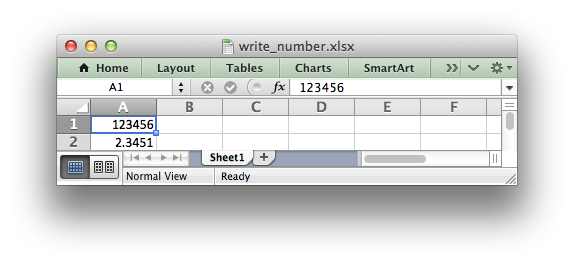The native data type for all numbers in Excel is a IEEE-754 64-bit double-precision floating point, which is also the default type used by `worksheet_write_number`.

The `format` parameter is used to apply formatting to the cell. This parameter can be `NULL` to indicate no formatting or it can be a Format object.

format_set_num_format(format, "\$#,##0.00");
worksheet_write_number(worksheet, 0, 0, 1234.567, format);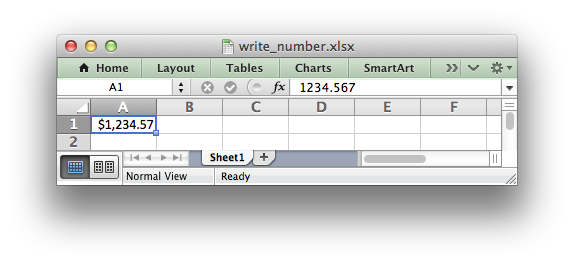Note
Excel doesn't support `NaN`, `Inf` or `-Inf` as a number value. If you are writing data that contains these values then your application should convert them to a string or handle them in some other way.
Examples
anatomy.c, array_formula.c, autofilter.c, chart.c, chart_area.c, chart_bar.c, chart_clustered.c, chart_column.c, chart_data_labels.c, chart_data_table.c, chart_data_tools.c, chart_doughnut.c, chart_fonts.c, chart_line.c, chart_pattern.c, chart_pie.c, chart_radar.c, chart_scatter.c, chart_styles.c, chartsheet.c, conditional_format1.c, conditional_format2.c, constant_memory.c, data_validate.c, dates_and_times01.c, demo.c, format_num_format.c, hello.c, outline.c, outline_collapsed.c, panes.c, tutorial1.c, tutorial2.c, and tutorial3.c.

## ◆ worksheet_write_string()

 lxw_error worksheet_write_string ( lxw_worksheet * worksheet, lxw_row_t row, lxw_col_t col, const char * string, lxw_format * format )
Parameters
 worksheet Pointer to a lxw_worksheet instance to be updated. row The zero indexed row number. col The zero indexed column number. string String to write to cell. format A pointer to a Format instance or NULL.
Returns
A lxw_error code.

The `worksheet_write_string()` function writes a string to the cell specified by `row` and `column`:

worksheet_write_string(worksheet, 0, 0, "This phrase is English!", NULL);The `format` parameter is used to apply formatting to the cell. This parameter can be `NULL` to indicate no formatting or it can be a Format object:

worksheet_write_string(worksheet, 0, 0, "This phrase is Bold!", format);Unicode strings are supported in UTF-8 encoding. This generally requires that your source file is UTF-8 encoded or that the data has been read from a UTF-8 source:

worksheet_write_string(worksheet, 0, 0, "Это фраза на русском!", NULL);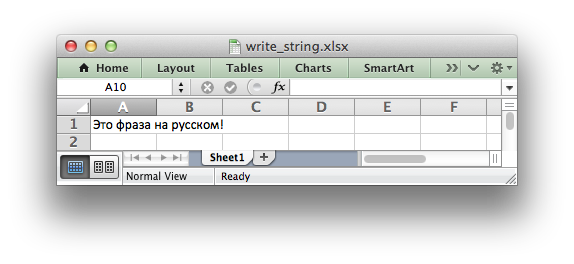Examples
anatomy.c, autofilter.c, chart_area.c, chart_bar.c, chart_clustered.c, chart_column.c, chart_data_labels.c, chart_data_table.c, chart_data_tools.c, chart_doughnut.c, chart_line.c, chart_pattern.c, chart_pie.c, chart_radar.c, chart_scatter.c, chartsheet.c, comments1.c, comments2.c, conditional_format2.c, data_validate.c, dates_and_times03.c, defined_name.c, demo.c, diagonal_border.c, doc_custom_properties.c, doc_properties.c, format_font.c, headers_footers.c, hello.c, hide_row_col.c, hide_sheet.c, hyperlinks.c, ignore_errors.c, images.c, macro.c, outline.c, outline_collapsed.c, panes.c, tab_colors.c, tutorial1.c, tutorial2.c, tutorial3.c, utf8.c, and worksheet_protection.c.

## ◆ worksheet_write_formula()

 lxw_error worksheet_write_formula ( lxw_worksheet * worksheet, lxw_row_t row, lxw_col_t col, const char * formula, lxw_format * format )
Parameters
 worksheet Pointer to a lxw_worksheet instance to be updated. row The zero indexed row number. col The zero indexed column number. formula Formula string to write to cell. format A pointer to a Format instance or NULL.
Returns
A lxw_error code.

The `worksheet_write_formula()` function writes a formula or function to the cell specified by `row` and `column`:

worksheet_write_formula(worksheet, 0, 0, "=B3 + 6", NULL);
worksheet_write_formula(worksheet, 1, 0, "=SIN(PI()/4)", NULL);
worksheet_write_formula(worksheet, 2, 0, "=SUM(A1:A2)", NULL);
worksheet_write_formula(worksheet, 3, 0, "=IF(A3>1,\"Yes\", \"No\")", NULL);
worksheet_write_formula(worksheet, 4, 0, "=AVERAGE(1, 2, 3, 4)", NULL);
worksheet_write_formula(worksheet, 5, 0, "=DATEVALUE(\"1-Jan-2013\")", NULL);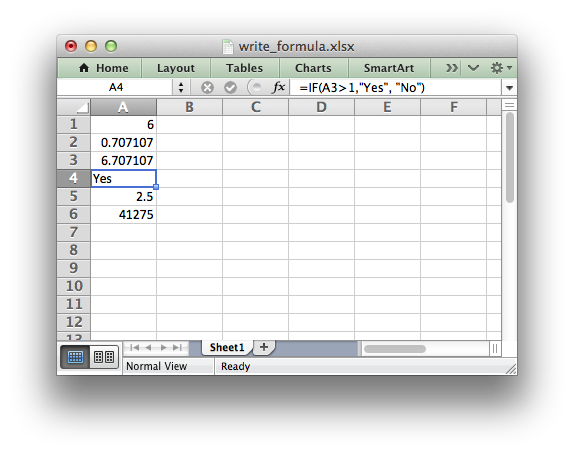The `format` parameter is used to apply formatting to the cell. This parameter can be `NULL` to indicate no formatting or it can be a Format object.

Libxlsxwriter doesn't calculate the value of a formula and instead stores a default value of `0`. The correct formula result is displayed in Excel, as shown in the example above, since it recalculates the formulas when it loads the file. For cases where this is an issue see the `worksheet_write_formula_num()` function and the discussion in that section.

Formulas must be written with the US style separator/range operator which is a comma (not semi-colon). Therefore a formula with multiple values should be written as follows:

// OK.
worksheet_write_formula(worksheet, 0, 0, "=SUM(1, 2, 3)", NULL);
worksheet_write_formula(worksheet, 1, 0, "=SUM(1; 2; 3)", NULL);

Examples
data_validate.c, defined_name.c, ignore_errors.c, outline.c, outline_collapsed.c, tutorial1.c, tutorial2.c, tutorial3.c, and worksheet_protection.c.

## ◆ worksheet_write_array_formula()

 lxw_error worksheet_write_array_formula ( lxw_worksheet * worksheet, lxw_row_t first_row, lxw_col_t first_col, lxw_row_t last_row, lxw_col_t last_col, const char * formula, lxw_format * format )
Parameters
 worksheet Pointer to a lxw_worksheet instance to be updated. first_row The first row of the range. (All zero indexed.) first_col The first column of the range. last_row The last row of the range. last_col The last col of the range. formula Array formula to write to cell. format A pointer to a Format instance or NULL.
Returns
A lxw_error code.

The `worksheet_write_array_formula()` function writes an array formula to a cell range. In Excel an array formula is a formula that performs a calculation on a set of values.

In Excel an array formula is indicated by a pair of braces around the formula: `{=SUM(A1:B1*A2:B2)}`.

Array formulas can return a single value or a range or values. For array formulas that return a range of values you must specify the range that the return values will be written to. This is why this function has `first_` and `last_` row/column parameters. The RANGE() macro can also be used to specify the range:

worksheet_write_array_formula(worksheet, 4, 0, 6, 0, "{=TREND(C5:C7,B5:B7)}", NULL);
// Same as above using the RANGE() macro.
worksheet_write_array_formula(worksheet, RANGE("A5:A7"), "{=TREND(C5:C7,B5:B7)}", NULL);

If the array formula returns a single value then the `first_` and `last_` parameters should be the same:

worksheet_write_array_formula(worksheet, 1, 0, 1, 0, "{=SUM(B1:C1*B2:C2)}", NULL);
worksheet_write_array_formula(worksheet, RANGE("A2:A2"), "{=SUM(B1:C1*B2:C2)}", NULL);
Examples
array_formula.c.

## ◆ worksheet_write_datetime()

 lxw_error worksheet_write_datetime ( lxw_worksheet * worksheet, lxw_row_t row, lxw_col_t col, lxw_datetime * datetime, lxw_format * format )
Parameters
 worksheet Pointer to a lxw_worksheet instance to be updated. row The zero indexed row number. col The zero indexed column number. datetime The datetime to write to the cell. format A pointer to a Format instance or NULL.
Returns
A lxw_error code.

The `worksheet_write_datetime()` function can be used to write a date or time to the cell specified by `row` and `column`:

#include "xlsxwriter.h"
int main() {
/* A datetime to display. */
lxw_datetime datetime = {2013, 2, 28, 12, 0, 0.0};
/* Create a new workbook and add a worksheet. */
lxw_workbook *workbook = workbook_new("date_and_times02.xlsx");
/* Add a format with date formatting. */
format_set_num_format(format, "mmm d yyyy hh:mm AM/PM");
worksheet_write_datetime(worksheet, 1, 0, &datetime, format); // Feb 28 2013 12:00 PM
return workbook_close(workbook);
}

The `format` parameter should be used to apply formatting to the cell using a Format object as shown above. Without a date format the datetime will appear as a number only.

Examples
dates_and_times02.c, dates_and_times03.c, and tutorial3.c.

## ◆ worksheet_write_url()

 lxw_error worksheet_write_url ( lxw_worksheet * worksheet, lxw_row_t row, lxw_col_t col, const char * url, lxw_format * format )
Parameters
 worksheet Pointer to a lxw_worksheet instance to be updated. row The zero indexed row number. col The zero indexed column number. url The url to write to the cell. format A pointer to a Format instance or NULL.
Returns
A lxw_error code.

The `worksheet_write_url()` function is used to write a URL/hyperlink to a worksheet cell specified by `row` and `column`.

worksheet_write_url(worksheet, 0, 0, "http://libxlsxwriter.github.io", NULL);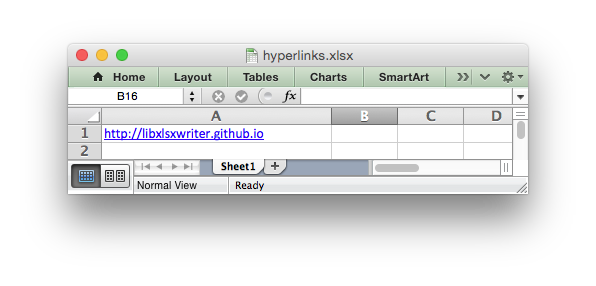The `format` parameter is used to apply formatting to the cell. This parameter can be `NULL`, in which case the default Excel blue underlined hyperlink style will be used. If required a user defined Format object can be used: underline:

The usual web style URI's are supported: `http://`, `https://`, `ftp://` and `mailto:` :

worksheet_write_url(worksheet, 0, 0, "ftp://www.python.org/", NULL);
worksheet_write_url(worksheet, 1, 0, "http://www.python.org/", NULL);
worksheet_write_url(worksheet, 2, 0, "https://www.python.org/", NULL);
worksheet_write_url(worksheet, 3, 0, "mailto:jmcnamara@cpan.org", NULL);

An Excel hyperlink is comprised of two elements: the displayed string and the non-displayed link. By default the displayed string is the same as the link. However, it is possible to overwrite it with any other `libxlsxwriter` type using the appropriate `worksheet_write_*()` function. The most common case is to overwrite the displayed link text with another string. To do this we must also match the default URL format using `workbook_get_default_url_format()`:

// Write a hyperlink with the default blue underline format.
worksheet_write_url(worksheet, 2, 0, "http://libxlsxwriter.github.io", NULL);
// Get the default url format.
// Overwrite the hyperlink with a user defined string and default format.
worksheet_write_string(worksheet, 2, 0, "Read the documentation.", url_format);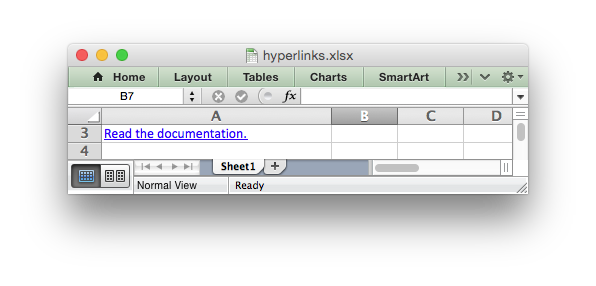Two local URIs are supported: `internal:` and `external:`. These are used for hyperlinks to internal worksheet references or external workbook and worksheet references:

worksheet_write_url(worksheet, 0, 0, "internal:Sheet2!A1", NULL);
worksheet_write_url(worksheet, 1, 0, "internal:Sheet2!B2", NULL);
worksheet_write_url(worksheet, 2, 0, "internal:Sheet2!A1:B2", NULL);
worksheet_write_url(worksheet, 3, 0, "internal:'Sales Data'!A1", NULL);
worksheet_write_url(worksheet, 4, 0, "external:c:\\temp\\foo.xlsx", NULL);
worksheet_write_url(worksheet, 5, 0, "external:c:\\foo.xlsx#Sheet2!A1", NULL);
worksheet_write_url(worksheet, 6, 0, "external:..\\foo.xlsx", NULL);
worksheet_write_url(worksheet, 7, 0, "external:..\\foo.xlsx#Sheet2!A1", NULL);
worksheet_write_url(worksheet, 8, 0, "external:\\\\NET\\share\\foo.xlsx", NULL);

Worksheet references are typically of the form `Sheet1!A1`. You can also link to a worksheet range using the standard Excel notation: `Sheet1!A1:B2`.

In external links the workbook and worksheet name must be separated by the `#` character:

worksheet_write_url(worksheet, 0, 0, "external:c:\\foo.xlsx#Sheet2!A1", NULL);

You can also link to a named range in the target worksheet: For example say you have a named range called `my_name` in the workbook `c:\temp\foo.xlsx` you could link to it as follows:

worksheet_write_url(worksheet, 0, 0, "external:c:\\temp\\foo.xlsx#my_name", NULL);

Excel requires that worksheet names containing spaces or non alphanumeric characters are single quoted as follows:

worksheet_write_url(worksheet, 0, 0, "internal:'Sales Data'!A1", NULL);

Links to network files are also supported. Network files normally begin with two back slashes as follows `\\NETWORK\etc`. In order to represent this in a C string literal the backslashes should be escaped:

worksheet_write_url(worksheet, 0, 0, "external:\\\\NET\\share\\foo.xlsx", NULL);

Alternatively, you can use Unix style forward slashes. These are translated internally to backslashes:

worksheet_write_url(worksheet, 0, 0, "external:c:/temp/foo.xlsx", NULL);
worksheet_write_url(worksheet, 1, 0, "external://NET/share/foo.xlsx", NULL);

Note:

libxlsxwriter will escape the following characters in URLs as required by Excel: `\s " < > \ [ ] ^ { }`. Existing URL `%xx` style escapes in the string are ignored to allow for user-escaped strings.

Note:

The maximum allowable URL length in recent versions of Excel is 2079 characters. In older versions of Excel (and libxlsxwriter <= 0.8.8) the limit was 255 characters.

Examples

## ◆ worksheet_write_boolean()

 lxw_error worksheet_write_boolean ( lxw_worksheet * worksheet, lxw_row_t row, lxw_col_t col, int value, lxw_format * format )
Parameters
 worksheet Pointer to a lxw_worksheet instance to be updated. row The zero indexed row number. col The zero indexed column number. value The boolean value to write to the cell. format A pointer to a Format instance or NULL.
Returns
A lxw_error code.

Write an Excel boolean to the cell specified by `row` and `column`:

worksheet_write_boolean(worksheet, 2, 2, 0, my_format);

## ◆ worksheet_write_blank()

 lxw_error worksheet_write_blank ( lxw_worksheet * worksheet, lxw_row_t row, lxw_col_t col, lxw_format * format )
Parameters
 worksheet Pointer to a lxw_worksheet instance to be updated. row The zero indexed row number. col The zero indexed column number. format A pointer to a Format instance or NULL.
Returns
A lxw_error code.

Write a blank cell specified by `row` and `column`:

worksheet_write_blank(worksheet, 1, 1, border_format);

This function is used to add formatting to a cell which doesn't contain a string or number value.

Excel differentiates between an "Empty" cell and a "Blank" cell. An Empty cell is a cell which doesn't contain data or formatting whilst a Blank cell doesn't contain data but does contain formatting. Excel stores Blank cells but ignores Empty cells.

As such, if you write an empty cell without formatting it is ignored.

## ◆ worksheet_write_formula_num()

 lxw_error worksheet_write_formula_num ( lxw_worksheet * worksheet, lxw_row_t row, lxw_col_t col, const char * formula, lxw_format * format, double result )
Parameters
 worksheet Pointer to a lxw_worksheet instance to be updated. row The zero indexed row number. col The zero indexed column number. formula Formula string to write to cell. format A pointer to a Format instance or NULL. result A user defined numeric result for the formula.
Returns
A lxw_error code.

The `worksheet_write_formula_num()` function writes a formula or Excel function to the cell specified by `row` and `column` with a user defined numeric result:

// Required as a workaround only.
worksheet_write_formula_num(worksheet, 0, 0, "=1 + 2", NULL, 3);

Libxlsxwriter doesn't calculate the value of a formula and instead stores the value `0` as the formula result. It then sets a global flag in the XLSX file to say that all formulas and functions should be recalculated when the file is opened.

This is the method recommended in the Excel documentation and in general it works fine with spreadsheet applications.

However, applications that don't have a facility to calculate formulas, such as Excel Viewer, or some mobile applications will only display the `0` results.

If required, the `worksheet_write_formula_num()` function can be used to specify a formula and its result.

This function is rarely required and is only provided for compatibility with some third party applications. For most applications the worksheet_write_formula() function is the recommended way of writing formulas.

## ◆ worksheet_write_formula_str()

 lxw_error worksheet_write_formula_str ( lxw_worksheet * worksheet, lxw_row_t row, lxw_col_t col, const char * formula, lxw_format * format, const char * result )
Parameters
 worksheet Pointer to a lxw_worksheet instance to be updated. row The zero indexed row number. col The zero indexed column number. formula Formula string to write to cell. format A pointer to a Format instance or NULL. result A user defined string result for the formula.
Returns
A lxw_error code.

The `worksheet_write_formula_str()` function writes a formula or Excel function to the cell specified by `row` and `column` with a user defined string result:

// The example formula is A & B -> AB.
worksheet_write_formula_str(worksheet, 0, 0, "=\"A\" & \"B\"", NULL, "AB");

The `worksheet_write_formula_str()` function is similar to the `worksheet_write_formula_num()` function except it writes a string result instead or a numeric result. See `worksheet_write_formula_num()` for more details on why/when these functions are required.

One place where the `worksheet_write_formula_str()` function may be required is to specify an empty result which will force a recalculation of the formula when loaded in LibreOffice.

worksheet_write_formula_str(worksheet, 0, 0, "=Sheet1!\$A\$1", NULL, "");

## ◆ worksheet_write_rich_string()

 lxw_error worksheet_write_rich_string ( lxw_worksheet * worksheet, lxw_row_t row, lxw_col_t col, lxw_rich_string_tuple * rich_string[], lxw_format * format )
Parameters
 worksheet Pointer to a lxw_worksheet instance to be updated. row The zero indexed row number. col The zero indexed column number. rich_string An array of format/string lxw_rich_string_tuple fragments. format A pointer to a Format instance or NULL.
Returns
A lxw_error code.

The `worksheet_write_rich_string()` function is used to write strings with multiple formats. For example to write the string 'This is bold and this is italic' you would use the following:

lxw_rich_string_tuple fragment11 = {.format = NULL, .string = "This is " };
lxw_rich_string_tuple fragment12 = {.format = bold, .string = "bold" };
lxw_rich_string_tuple fragment13 = {.format = NULL, .string = " and this is "};
lxw_rich_string_tuple fragment14 = {.format = italic, .string = "italic" };
lxw_rich_string_tuple *rich_string1[] = {&fragment11, &fragment12,
&fragment13, &fragment14, NULL};
worksheet_write_rich_string(worksheet, CELL("A1"), rich_string1, NULL);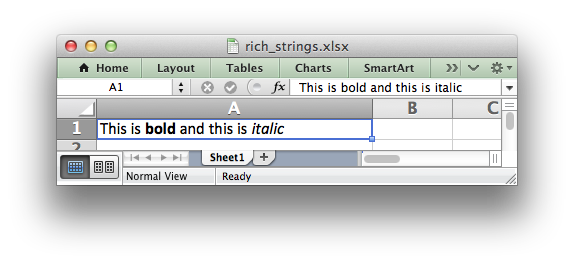The basic rule is to break the string into fragments and put a lxw_format object before the fragment that you want to format. So if we look at the above example again:

This is bold and this is italic

The would be broken down into 4 fragments:

``` default: |This is |
bold:    |bold|
default: | and this is |
italic:  |italic|
```

This in then converted to the lxw_rich_string_tuple fragments shown in the example above. For the default format we use `NULL`.

The fragments are passed to `worksheet_write_rich_string()` as a `NULL` terminated array:

lxw_rich_string_tuple *rich_string1[] = {&fragment11, &fragment12,
&fragment13, &fragment14, NULL};
worksheet_write_rich_string(worksheet, CELL("A1"), rich_string1, NULL);

Note: Excel doesn't allow the use of two consecutive formats in a rich string or an empty string fragment. For either of these conditions a warning is raised and the input to `worksheet_write_rich_string()` is ignored.

Examples
merge_rich_string.c, and rich_strings.c.

## ◆ worksheet_write_comment()

 lxw_error worksheet_write_comment ( lxw_worksheet * worksheet, lxw_row_t row, lxw_col_t col, const char * string )
Parameters
 worksheet Pointer to a lxw_worksheet instance to be updated. row The zero indexed row number. col The zero indexed column number. string The comment string to be written.
Returns
A lxw_error code.

The `worksheet_write_comment()` function is used to add a comment to a cell. A comment is indicated in Excel by a small red triangle in the upper right-hand corner of the cell. Moving the cursor over the red triangle will reveal the comment.

The following example shows how to add a comment to a cell:

worksheet_write_comment(worksheet, 0, 0, "This is a comment");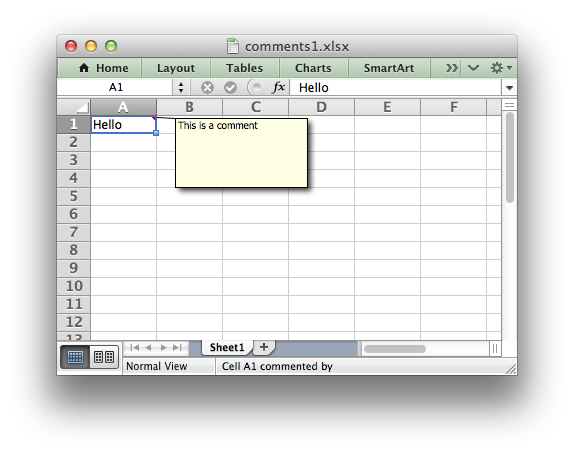Examples

## ◆ worksheet_write_comment_opt()

 lxw_error worksheet_write_comment_opt ( lxw_worksheet * worksheet, lxw_row_t row, lxw_col_t col, const char * string, lxw_comment_options * options )
Parameters
 worksheet Pointer to a lxw_worksheet instance to be updated. row The zero indexed row number. col The zero indexed column number. string The comment string to be written. options lxw_comment_options to control position and format of the comment.
Returns
A lxw_error code.

The `worksheet_write_comment_opt()` function is used to add a comment to a cell with option that control the position, format and metadata of the comment. A comment is indicated in Excel by a small red triangle in the upper right-hand corner of the cell. Moving the cursor over the red triangle will reveal the comment.

The following example shows how to add a comment to a cell with options:

worksheet_write_comment_opt(worksheet, CELL("C6"), "Hello.", &options);

The following options are available in lxw_comment_options:

• `author`
• `visible`
• `width`
• `height`
• `x_scale`
• `y_scale`
• `color`
• `font_name`
• `font_size`
• `start_row`
• `start_col`
• `x_offset`
• `y_offset`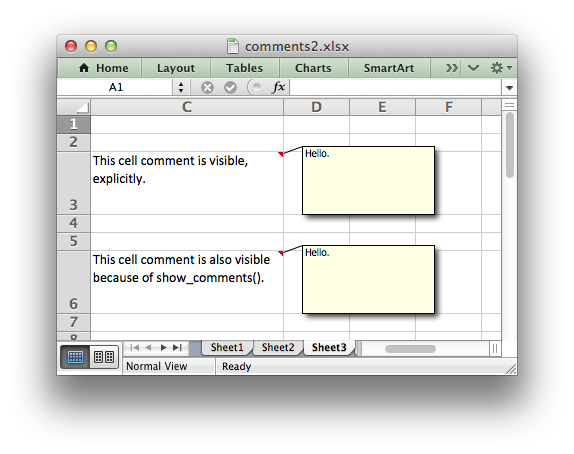Comment options are explained in detail in the Setting Comment Properties section of the docs.

Examples

## ◆ worksheet_set_row()

 lxw_error worksheet_set_row ( lxw_worksheet * worksheet, lxw_row_t row, double height, lxw_format * format )
Parameters
 worksheet Pointer to a lxw_worksheet instance to be updated. row The zero indexed row number. height The row height. format A pointer to a Format instance or NULL.

The `worksheet_set_row()` function is used to change the default properties of a row. The most common use for this function is to change the height of a row:

// Set the height of Row 1 to 20.
worksheet_set_row(worksheet, 0, 20, NULL);

The other common use for `worksheet_set_row()` is to set the a Format for all cells in the row:

// Set the header row to bold.
worksheet_set_row(worksheet, 0, 15, bold);

If you wish to set the format of a row without changing the height you can pass the default row height of LXW_DEF_ROW_HEIGHT = 15:

worksheet_set_row(worksheet, 0, LXW_DEF_ROW_HEIGHT, format);
worksheet_set_row(worksheet, 0, 15, format); // Same as above.

The `format` parameter will be applied to any cells in the row that don't have a format. As with Excel the row format is overridden by an explicit cell format. For example:

// Row 1 has format1.
worksheet_set_row(worksheet, 0, 15, format1);
// Cell A1 in Row 1 defaults to format1.
worksheet_write_string(worksheet, 0, 0, "Hello", NULL);
// Cell B1 in Row 1 keeps format2.
worksheet_write_string(worksheet, 0, 1, "Hello", format2);
Examples
comments2.c, data_validate.c, hide_row_col.c, merge_range.c, outline.c, outline_collapsed.c, and panes.c.

## ◆ worksheet_set_row_opt()

 lxw_error worksheet_set_row_opt ( lxw_worksheet * worksheet, lxw_row_t row, double height, lxw_format * format, lxw_row_col_options * options )
Parameters
 worksheet Pointer to a lxw_worksheet instance to be updated. row The zero indexed row number. height The row height. format A pointer to a Format instance or NULL. options Optional row parameters: hidden, level, collapsed.

The `worksheet_set_row_opt()` function is the same as `worksheet_set_row()` with an additional `options` parameter.

The `options` parameter is a lxw_row_col_options struct. It has the following members:

• `hidden`
• `level`
• `collapsed`

The `"hidden"` option is used to hide a row. This can be used, for example, to hide intermediary steps in a complicated calculation:

lxw_row_col_options options1 = {.hidden = 1, .level = 0, .collapsed = 0};
// Hide the fourth and fifth (zero indexed) rows.
worksheet_set_row_opt(worksheet, 3, LXW_DEF_ROW_HEIGHT, NULL, &options1);
worksheet_set_row_opt(worksheet, 4, LXW_DEF_ROW_HEIGHT, NULL, &options1);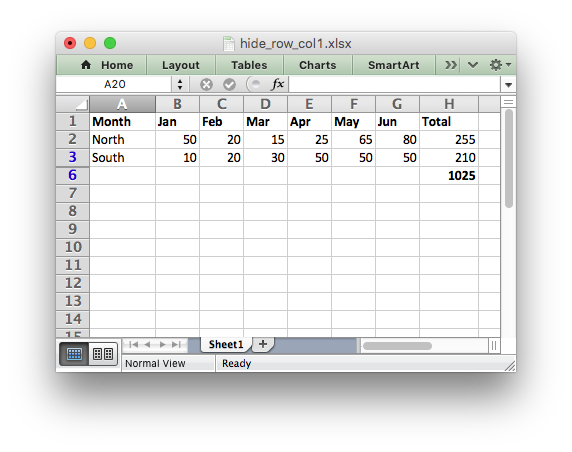The `"hidden"`, `"level"`, and `"collapsed"`, options can also be used to create Outlines and Grouping. See Working with Outlines and Grouping.

// The option structs with the outline level set.
lxw_row_col_options options1 = {.hidden = 0, .level = 2, .collapsed = 0};
lxw_row_col_options options2 = {.hidden = 0, .level = 1, .collapsed = 0};
// Set the row options with the outline level.
worksheet_set_row_opt(worksheet, 1, LXW_DEF_ROW_HEIGHT, NULL, &options1);
worksheet_set_row_opt(worksheet, 2, LXW_DEF_ROW_HEIGHT, NULL, &options1);
worksheet_set_row_opt(worksheet, 3, LXW_DEF_ROW_HEIGHT, NULL, &options1);
worksheet_set_row_opt(worksheet, 4, LXW_DEF_ROW_HEIGHT, NULL, &options1);
worksheet_set_row_opt(worksheet, 5, LXW_DEF_ROW_HEIGHT, NULL, &options2);
worksheet_set_row_opt(worksheet, 6, LXW_DEF_ROW_HEIGHT, NULL, &options1);
worksheet_set_row_opt(worksheet, 7, LXW_DEF_ROW_HEIGHT, NULL, &options1);
worksheet_set_row_opt(worksheet, 8, LXW_DEF_ROW_HEIGHT, NULL, &options1);
worksheet_set_row_opt(worksheet, 9, LXW_DEF_ROW_HEIGHT, NULL, &options1);
worksheet_set_row_opt(worksheet, 10, LXW_DEF_ROW_HEIGHT, NULL, &options2);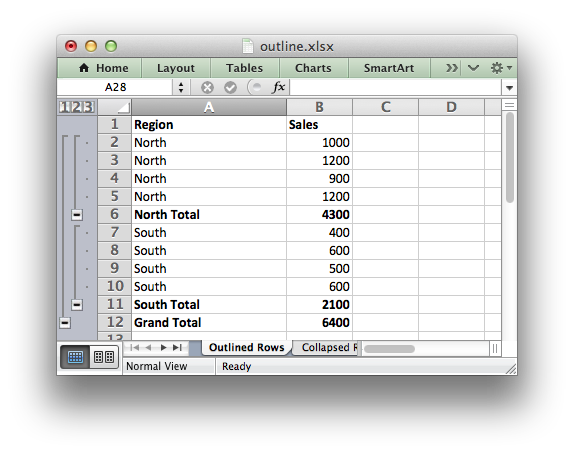Examples
outline.c, and outline_collapsed.c.

## ◆ worksheet_set_column()

 lxw_error worksheet_set_column ( lxw_worksheet * worksheet, lxw_col_t first_col, lxw_col_t last_col, double width, lxw_format * format )
Parameters
 worksheet Pointer to a lxw_worksheet instance to be updated. first_col The zero indexed first column. last_col The zero indexed last column. width The width of the column(s). format A pointer to a Format instance or NULL.

The `worksheet_set_column()` function can be used to change the default properties of a single column or a range of columns:

// Width of columns B:D set to 30.
worksheet_set_column(worksheet, 1, 3, 30, NULL);

If `worksheet_set_column()` is applied to a single column the value of `first_col` and `last_col` should be the same:

// Width of column B set to 30.
worksheet_set_column(worksheet, 1, 1, 30, NULL);

It is also possible, and generally clearer, to specify a column range using the form of `COLS()` macro:

worksheet_set_column(worksheet, 4, 4, 20, NULL);
worksheet_set_column(worksheet, 5, 8, 30, NULL);
// Same as the examples above but clearer.
worksheet_set_column(worksheet, COLS("E:E"), 20, NULL);
worksheet_set_column(worksheet, COLS("F:H"), 30, NULL);

The `width` parameter sets the column width in the same units used by Excel which is: the number of characters in the default font. The default width is 8.43 in the default font of Calibri 11. The actual relationship between a string width and a column width in Excel is complex. See the following explanation of column widths from the Microsoft support documentation for more details.

There is no way to specify "AutoFit" for a column in the Excel file format. This feature is only available at runtime from within Excel. It is possible to simulate "AutoFit" in your application by tracking the maximum width of the data in the column as your write it and then adjusting the column width at the end.

As usual the format.h `format` parameter is optional. If you wish to set the format without changing the width you can pass a default column width of LXW_DEF_COL_WIDTH = 8.43:

// Set the first column to bold.
worksheet_set_column(worksheet, 0, 0, LXW_DEF_COL_WIDTH, bold);

The `format` parameter will be applied to any cells in the column that don't have a format. For example:

// Column 1 has format1.
worksheet_set_column(worksheet, COLS("A:A"), 8.43, format1);
// Cell A1 in column 1 defaults to format1.
worksheet_write_string(worksheet, 0, 0, "Hello", NULL);
// Cell A2 in column 1 keeps format2.
worksheet_write_string(worksheet, 1, 0, "Hello", format2);

As in Excel a row format takes precedence over a default column format:

// Row 1 has format1.
worksheet_set_row(worksheet, 0, 15, format1);
// Col 1 has format2.
worksheet_set_column(worksheet, COLS("A:A"), 8.43, format2);
// Cell A1 defaults to format1, the row format.
worksheet_write_string(worksheet, 0, 0, "Hello", NULL);
// Cell A2 keeps format2, the column format.
worksheet_write_string(worksheet, 1, 0, "Hello", NULL);
Examples
anatomy.c, comments2.c, data_validate.c, dates_and_times01.c, dates_and_times02.c, dates_and_times03.c, defined_name.c, demo.c, doc_custom_properties.c, doc_properties.c, format_font.c, format_num_format.c, headers_footers.c, hide_sheet.c, hyperlinks.c, ignore_errors.c, images.c, merge_range.c, outline.c, outline_collapsed.c, panes.c, rich_strings.c, tutorial3.c, and worksheet_protection.c.

## ◆ worksheet_set_column_opt()

 lxw_error worksheet_set_column_opt ( lxw_worksheet * worksheet, lxw_col_t first_col, lxw_col_t last_col, double width, lxw_format * format, lxw_row_col_options * options )
Parameters
 worksheet Pointer to a lxw_worksheet instance to be updated. first_col The zero indexed first column. last_col The zero indexed last column. width The width of the column(s). format A pointer to a Format instance or NULL. options Optional row parameters: hidden, level, collapsed.

The `worksheet_set_column_opt()` function is the same as `worksheet_set_column()` with an additional `options` parameter.

The `options` parameter is a lxw_row_col_options struct. It has the following members:

• `hidden`
• `level`
• `collapsed`

The `"hidden"` option is used to hide a column. This can be used, for example, to hide intermediary steps in a complicated calculation:

lxw_row_col_options options1 = {.hidden = 1, .level = 0, .collapsed = 0};
worksheet_set_column_opt(worksheet, COLS("D:E"), LXW_DEF_COL_WIDTH, NULL, &options1);The `"hidden"`, `"level"`, and `"collapsed"`, options can also be used to create Outlines and Grouping. See Working with Outlines and Grouping.

lxw_row_col_options options1 = {.hidden = 0, .level = 1, .collapsed = 0};
worksheet_set_column_opt(worksheet, COLS("B:G"), 5, NULL, &options1);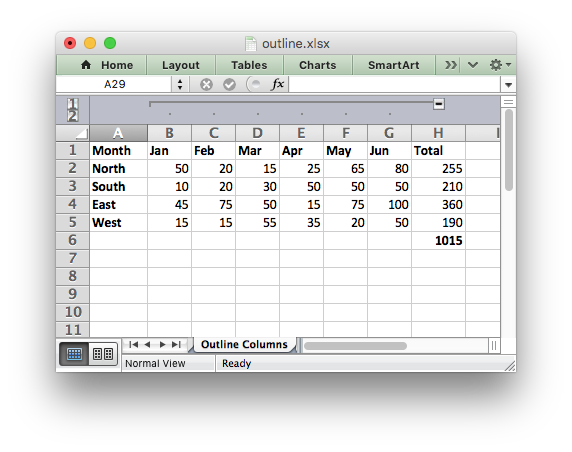Examples
hide_row_col.c, outline.c, and outline_collapsed.c.

## ◆ worksheet_insert_image()

 lxw_error worksheet_insert_image ( lxw_worksheet * worksheet, lxw_row_t row, lxw_col_t col, const char * filename )
Parameters
 worksheet Pointer to a lxw_worksheet instance to be updated. row The zero indexed row number. col The zero indexed column number. filename The image filename, with path if required.
Returns
A lxw_error code.

This function can be used to insert a image into a worksheet. The image can be in PNG, JPEG or BMP format:

worksheet_insert_image(worksheet, 2, 1, "logo.png");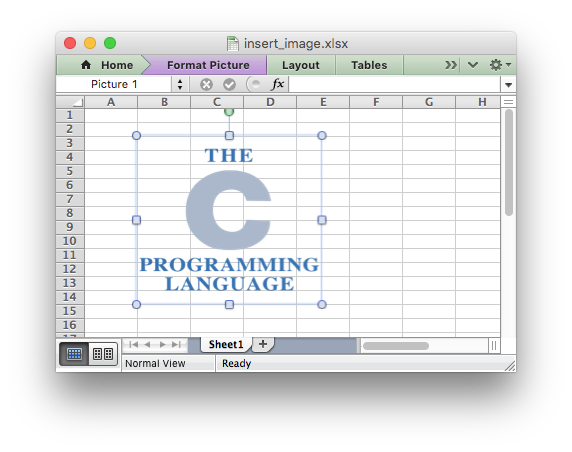The `worksheet_insert_image_opt()` function takes additional optional parameters to position and scale the image, see below.

Note: The scaling of a image may be affected if is crosses a row that has its default height changed due to a font that is larger than the default font size or that has text wrapping turned on. To avoid this you should explicitly set the height of the row using `worksheet_set_row()` if it crosses an inserted image. See Working with Object Positioning.

BMP images are only supported for backward compatibility. In general it is best to avoid BMP images since they aren't compressed. If used, BMP images must be 24 bit, true color, bitmaps.

Examples
demo.c, and images.c.

## ◆ worksheet_insert_image_opt()

 lxw_error worksheet_insert_image_opt ( lxw_worksheet * worksheet, lxw_row_t row, lxw_col_t col, const char * filename, lxw_image_options * options )
Parameters
 worksheet Pointer to a lxw_worksheet instance to be updated. row The zero indexed row number. col The zero indexed column number. filename The image filename, with path if required. options Optional image parameters.
Returns
A lxw_error code.

The `worksheet_insert_image_opt()` function is like `worksheet_insert_image()` function except that it takes an optional lxw_image_options struct to scale and position the image:

lxw_image_options options = {.x_offset = 30, .y_offset = 10,
.x_scale = 0.5, .y_scale = 0.5};
worksheet_insert_image_opt(worksheet, 2, 1, "logo.png", &options);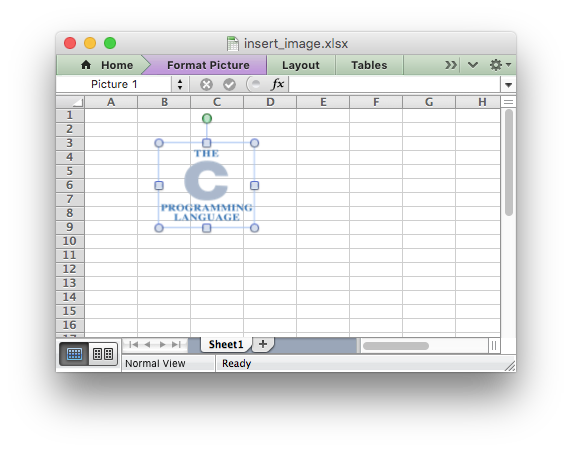The `url` field of lxw_image_options can be use to used to add a hyperlink to an image:

lxw_image_options options = {.url = "https://github.com/jmcnamara"};
worksheet_insert_image_opt(worksheet, 3, 1, "logo.png", &options);

The supported URL formats are the same as those supported by the `worksheet_write_url()` method and the same rules/limits apply.

The `tip` field of lxw_image_options can be use to used to add a mouseover tip to the hyperlink:

lxw_image_options options = {.url = "https://github.com/jmcnamara",
.tip = "GitHub"};
worksheet_insert_image_opt(worksheet, 4, 1, "logo.png", &options);
Note
See the notes about row scaling and BMP images in `worksheet_insert_image()` above.
Examples
images.c.

## ◆ worksheet_insert_image_buffer()

 lxw_error worksheet_insert_image_buffer ( lxw_worksheet * worksheet, lxw_row_t row, lxw_col_t col, const unsigned char * image_buffer, size_t image_size )
Parameters
 worksheet Pointer to a lxw_worksheet instance to be updated. row The zero indexed row number. col The zero indexed column number. image_buffer Pointer to an array of bytes that holds the image data. image_size The size of the array of bytes.
Returns
A lxw_error code.

This function can be used to insert a image into a worksheet from a memory buffer:

worksheet_insert_image_buffer(worksheet, CELL("B3"), image_buffer, image_size);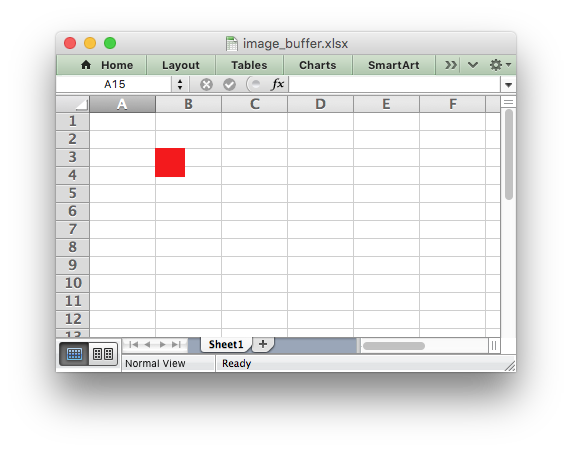The buffer should be a pointer to an array of unsigned char data with a specified size.

See `worksheet_insert_image()` for details about the supported image formats, and other image features.

Examples
image_buffer.c.

## ◆ worksheet_insert_image_buffer_opt()

 lxw_error worksheet_insert_image_buffer_opt ( lxw_worksheet * worksheet, lxw_row_t row, lxw_col_t col, const unsigned char * image_buffer, size_t image_size, lxw_image_options * options )
Parameters
 worksheet Pointer to a lxw_worksheet instance to be updated. row The zero indexed row number. col The zero indexed column number. image_buffer Pointer to an array of bytes that holds the image data. image_size The size of the array of bytes. options Optional image parameters.
Returns
A lxw_error code.

The `worksheet_insert_image_buffer_opt()` function is like `worksheet_insert_image_buffer()` function except that it takes an optional lxw_image_options struct to scale and position the image:

lxw_image_options options = {.x_offset = 32, .y_offset = 4,
.x_scale = 2, .y_scale = 1};
worksheet_insert_image_buffer_opt(worksheet, CELL("B3"), image_buffer, image_size, &options);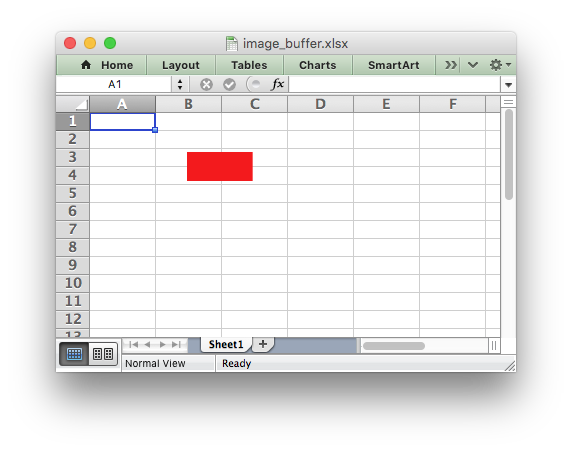The buffer should be a pointer to an array of unsigned char data with a specified size.

See `worksheet_insert_image_buffer_opt()` for details about the supported image formats, and other image options.

Examples
image_buffer.c.

## ◆ worksheet_insert_chart()

 lxw_error worksheet_insert_chart ( lxw_worksheet * worksheet, lxw_row_t row, lxw_col_t col, lxw_chart * chart )
Parameters
 worksheet Pointer to a lxw_worksheet instance to be updated. row The zero indexed row number. col The zero indexed column number. chart A lxw_chart object created via workbook_add_chart().
Returns
A lxw_error code.

The `worksheet_insert_chart()` function can be used to insert a chart into a worksheet. The chart object must be created first using the `workbook_add_chart()` function and configured using the chart.h functions.

// Create a chart object.
// Add a data series to the chart.
// Insert the chart into the worksheet.
worksheet_insert_chart(worksheet, 0, 2, chart);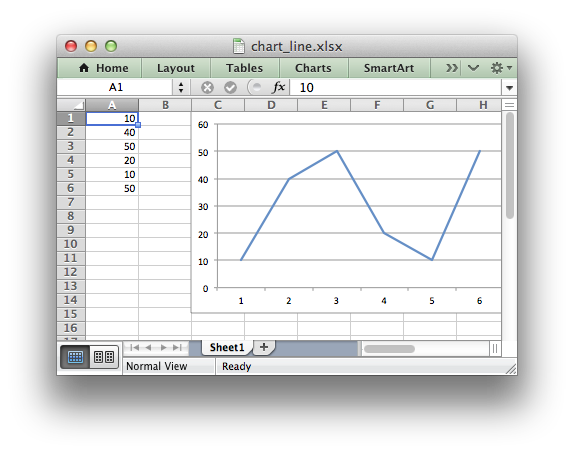Note:

A chart may only be inserted into a worksheet once. If several similar charts are required then each one must be created separately with `worksheet_insert_chart()`.

Examples
chart.c, chart_area.c, chart_bar.c, chart_clustered.c, chart_column.c, chart_data_table.c, chart_data_tools.c, chart_doughnut.c, chart_fonts.c, chart_line.c, chart_pattern.c, chart_pie.c, chart_radar.c, chart_scatter.c, and chart_styles.c.

## ◆ worksheet_insert_chart_opt()

 lxw_error worksheet_insert_chart_opt ( lxw_worksheet * worksheet, lxw_row_t row, lxw_col_t col, lxw_chart * chart, lxw_chart_options * user_options )
Parameters
 worksheet Pointer to a lxw_worksheet instance to be updated. row The zero indexed row number. col The zero indexed column number. chart A lxw_chart object created via workbook_add_chart(). user_options Optional chart parameters.
Returns
A lxw_error code.

The `worksheet_insert_chart_opt()` function is like `worksheet_insert_chart()` function except that it takes an optional lxw_chart_options struct to scale and position the chart:

lxw_chart_options options = {.x_offset = 30, .y_offset = 10,
.x_scale = 0.5, .y_scale = 0.75};
worksheet_insert_chart_opt(worksheet, 0, 2, chart, &options);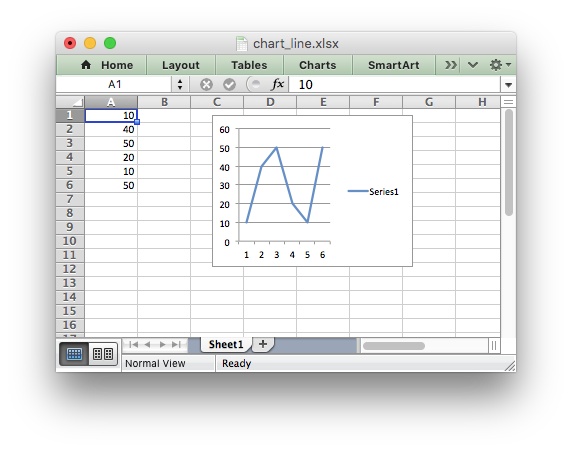Examples
chart_data_labels.c.

## ◆ worksheet_merge_range()

 lxw_error worksheet_merge_range ( lxw_worksheet * worksheet, lxw_row_t first_row, lxw_col_t first_col, lxw_row_t last_row, lxw_col_t last_col, const char * string, lxw_format * format )
Parameters
 worksheet Pointer to a lxw_worksheet instance to be updated. first_row The first row of the range. (All zero indexed.) first_col The first column of the range. last_row The last row of the range. last_col The last col of the range. string String to write to the merged range. format A pointer to a Format instance or NULL.
Returns
A lxw_error code.

The `worksheet_merge_range()` function allows cells to be merged together so that they act as a single area.

Excel generally merges and centers cells at same time. To get similar behavior with libxlsxwriter you need to apply a Format object with the appropriate alignment:

worksheet_merge_range(worksheet, 1, 1, 1, 3, "Merged Range", merge_format);

It is possible to apply other formatting to the merged cells as well:

format_set_bold (merge_format);
format_set_bg_color(merge_format, 0xD7E4BC);
worksheet_merge_range(worksheet, 2, 1, 3, 3, "Merged Range", merge_format);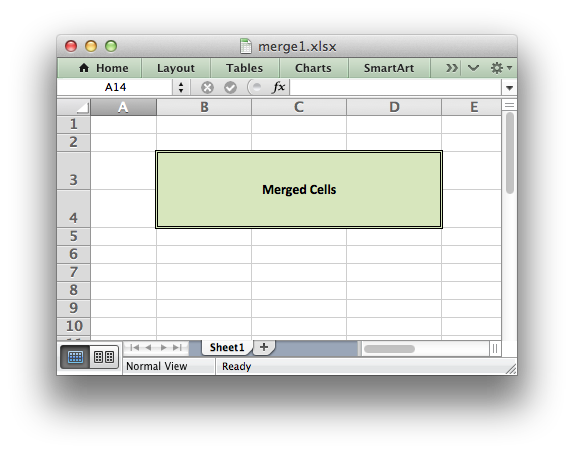The `worksheet_merge_range()` function writes a `char*` string using `worksheet_write_string()`. In order to write other data types, such as a number or a formula, you can overwrite the first cell with a call to one of the other write functions. The same Format should be used as was used in the merged range.

// First write a range with a blank string.
worksheet_merge_range (worksheet, 1, 1, 1, 3, "", format);
// Then overwrite the first cell with a number.
worksheet_write_number(worksheet, 1, 1, 123, format);
Note
Merged ranges generally don't work in libxlsxwriter when the Workbook lxw_workbook_options `constant_memory` mode is enabled.
Examples
merge_range.c, and merge_rich_string.c.

## ◆ worksheet_autofilter()

 lxw_error worksheet_autofilter ( lxw_worksheet * worksheet, lxw_row_t first_row, lxw_col_t first_col, lxw_row_t last_row, lxw_col_t last_col )
Parameters
 worksheet Pointer to a lxw_worksheet instance to be updated. first_row The first row of the range. (All zero indexed.) first_col The first column of the range. last_row The last row of the range. last_col The last col of the range.
Returns
A lxw_error code.

The `worksheet_autofilter()` function allows an autofilter to be added to a worksheet.

An autofilter is a way of adding drop down lists to the headers of a 2D range of worksheet data. This allows users to filter the data based on simple criteria so that some data is shown and some is hidden.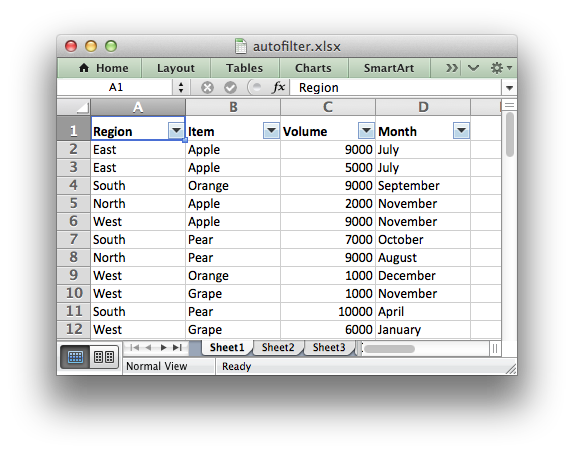To add an autofilter to a worksheet:

worksheet_autofilter(worksheet, 0, 0, 50, 3);
// Same as above using the RANGE() macro.
worksheet_autofilter(worksheet, RANGE("A1:D51"));

Note: it isn't currently possible to apply filter conditions to the autofilter.

Examples
autofilter.c.

## ◆ worksheet_data_validation_cell()

 lxw_error worksheet_data_validation_cell ( lxw_worksheet * worksheet, lxw_row_t row, lxw_col_t col, lxw_data_validation * validation )
Parameters
 worksheet Pointer to a lxw_worksheet instance to be updated. row The zero indexed row number. col The zero indexed column number. validation A lxw_data_validation object to control the validation.
Returns
A lxw_error code.

The `worksheet_data_validation_cell()` function is used to construct an Excel data validation or to limit the user input to a dropdown list of values:

lxw_data_validation *data_validation = calloc(1, sizeof(lxw_data_validation));
data_validation->minimum_number = 1;
data_validation->maximum_number = 10;
worksheet_data_validation_cell(worksheet, 2, 1, data_validation);
// Same as above with the CELL() macro.
worksheet_data_validation_cell(worksheet, CELL("B3"), data_validation);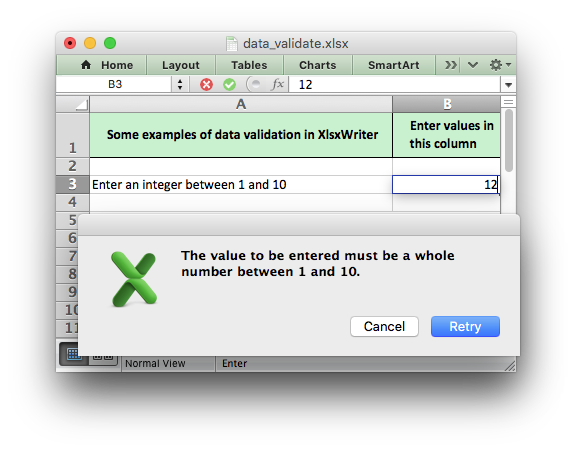Data validation and the various options of lxw_data_validation are described in more detail in Working with Data Validation.

Examples
data_validate.c.

## ◆ worksheet_data_validation_range()

 lxw_error worksheet_data_validation_range ( lxw_worksheet * worksheet, lxw_row_t first_row, lxw_col_t first_col, lxw_row_t last_row, lxw_col_t last_col, lxw_data_validation * validation )
Parameters
 worksheet Pointer to a lxw_worksheet instance to be updated. first_row The first row of the range. (All zero indexed.) first_col The first column of the range. last_row The last row of the range. last_col The last col of the range. validation A lxw_data_validation object to control the validation.
Returns
A lxw_error code.

The `worksheet_data_validation_range()` function is the same as the `worksheet_data_validation_cell()`, see above, except the data validation is applied to a range of cells:

lxw_data_validation *data_validation = calloc(1, sizeof(lxw_data_validation));
data_validation->minimum_number = 1;
data_validation->maximum_number = 10;
worksheet_data_validation_range(worksheet, 2, 1, 4, 1, data_validation);
// Same as above with the RANGE() macro.
worksheet_data_validation_range(worksheet, RANGE("B3:B5"), data_validation);

Data validation and the various options of lxw_data_validation are described in more detail in Working with Data Validation.

## ◆ worksheet_conditional_format_cell()

 lxw_error worksheet_conditional_format_cell ( lxw_worksheet * worksheet, lxw_row_t row, lxw_col_t col, lxw_conditional_format * conditional_format )
Parameters
 worksheet Pointer to a lxw_worksheet instance to be updated. row The zero indexed row number. col The zero indexed column number. conditional_format A lxw_conditional_format object to control the conditional format.
Returns
A lxw_error code.

The `worksheet_conditional_format_cell()` function is used to set a conditional format for a cell in a worksheet:

conditional_format->type = LXW_CONDITIONAL_TYPE_CELL;
conditional_format->value = 50;
conditional_format->format = format1;
worksheet_conditional_format_cell(worksheet, CELL("A1"), conditional_format);

The conditional format parameters is specified in lxw_conditional_format.

See Working with Conditional Formatting for full details.

## ◆ worksheet_conditional_format_range()

 lxw_error worksheet_conditional_format_range ( lxw_worksheet * worksheet, lxw_row_t first_row, lxw_col_t first_col, lxw_row_t last_row, lxw_col_t last_col, lxw_conditional_format * conditional_format )
Parameters
 worksheet Pointer to a lxw_worksheet instance to be updated. first_row The first row of the range. (All zero indexed.) first_col The first column of the range. last_row The last row of the range. last_col The last col of the range. conditional_format A lxw_conditional_format object to control the conditional format.
Returns
A lxw_error code.

The `worksheet_conditional_format_cell()` function is used to set a conditional format for a range of cells in a worksheet:

conditional_format->type = LXW_CONDITIONAL_TYPE_CELL;
conditional_format->value = 50;
conditional_format->format = format1;
worksheet_conditional_format_range(worksheet1, RANGE("B3:K12"), conditional_format);
conditional_format->type = LXW_CONDITIONAL_TYPE_CELL;
conditional_format->criteria = LXW_CONDITIONAL_CRITERIA_LESS_THAN;
conditional_format->value = 50;
conditional_format->format = format2;
worksheet_conditional_format_range(worksheet1, RANGE("B3:K12"), conditional_format);

Output: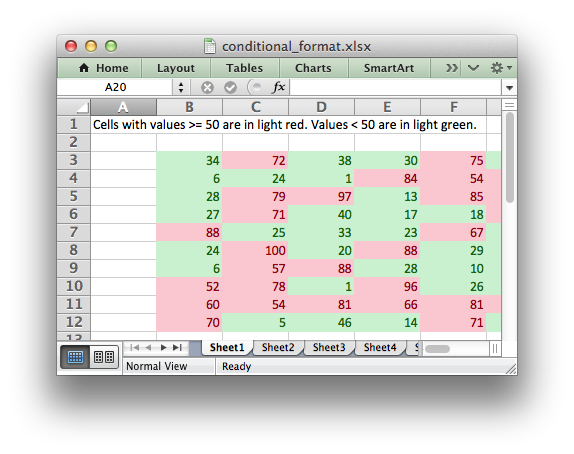The conditional format parameters is specified in lxw_conditional_format.

See Working with Conditional Formatting for full details.

Examples
conditional_format1.c, and conditional_format2.c.

## ◆ worksheet_activate()

 void worksheet_activate ( lxw_worksheet * worksheet )
Parameters
 worksheet Pointer to a lxw_worksheet instance to be updated.

The `worksheet_activate()` function is used to specify which worksheet is initially visible in a multi-sheet workbook:

worksheet_activate(worksheet3);More than one worksheet can be selected via the `worksheet_select()` function, see below, however only one worksheet can be active.

The default active worksheet is the first worksheet.

## ◆ worksheet_select()

 void worksheet_select ( lxw_worksheet * worksheet )
Parameters
 worksheet Pointer to a lxw_worksheet instance to be updated.

The `worksheet_select()` function is used to indicate that a worksheet is selected in a multi-sheet workbook:

worksheet_activate(worksheet1);
worksheet_select(worksheet2);
worksheet_select(worksheet3);

A selected worksheet has its tab highlighted. Selecting worksheets is a way of grouping them together so that, for example, several worksheets could be printed in one go. A worksheet that has been activated via the `worksheet_activate()` function will also appear as selected.

## ◆ worksheet_hide()

 void worksheet_hide ( lxw_worksheet * worksheet )
Parameters
 worksheet Pointer to a lxw_worksheet instance to be updated.

The `worksheet_hide()` function is used to hide a worksheet:

worksheet_hide(worksheet2);

You may wish to hide a worksheet in order to avoid confusing a user with intermediate data or calculations.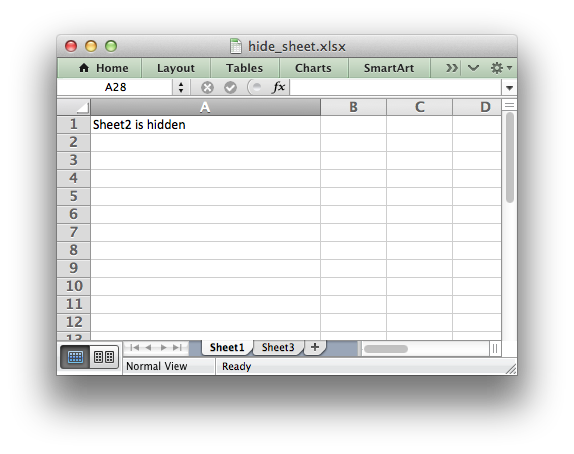A hidden worksheet can not be activated or selected so this function is mutually exclusive with the `worksheet_activate()` and `worksheet_select()` functions. In addition, since the first worksheet will default to being the active worksheet, you cannot hide the first worksheet without activating another sheet:

worksheet_activate(worksheet2);
worksheet_hide(worksheet1);
Examples
hide_sheet.c.

## ◆ worksheet_set_first_sheet()

 void worksheet_set_first_sheet ( lxw_worksheet * worksheet )
Parameters
 worksheet Pointer to a lxw_worksheet instance to be updated.

The `worksheet_activate()` function determines which worksheet is initially selected. However, if there are a large number of worksheets the selected worksheet may not appear on the screen. To avoid this you can select the leftmost visible worksheet tab using `worksheet_set_first_sheet()`:

worksheet_set_first_sheet(worksheet19); // First visible worksheet tab.
worksheet_activate(worksheet20); // First visible worksheet.

This function is not required very often. The default value is the first worksheet.

## ◆ worksheet_freeze_panes()

 void worksheet_freeze_panes ( lxw_worksheet * worksheet, lxw_row_t row, lxw_col_t col )
Parameters
 worksheet Pointer to a lxw_worksheet instance to be updated. row The cell row (zero indexed). col The cell column (zero indexed).

The `worksheet_freeze_panes()` function can be used to divide a worksheet into horizontal or vertical regions known as panes and to "freeze" these panes so that the splitter bars are not visible.

The parameters `row` and `col` are used to specify the location of the split. It should be noted that the split is specified at the top or left of a cell and that the function uses zero based indexing. Therefore to freeze the first row of a worksheet it is necessary to specify the split at row 2 (which is 1 as the zero-based index).

You can set one of the `row` and `col` parameters as zero if you do not want either a vertical or horizontal split.

Examples:

worksheet_freeze_panes(worksheet1, 1, 0); // Freeze the first row.
worksheet_freeze_panes(worksheet2, 0, 1); // Freeze the first column.
worksheet_freeze_panes(worksheet3, 1, 1); // Freeze first row/column.
Examples
panes.c.

## ◆ worksheet_split_panes()

 void worksheet_split_panes ( lxw_worksheet * worksheet, double vertical, double horizontal )
Parameters
 worksheet Pointer to a lxw_worksheet instance to be updated. vertical The position for the vertical split. horizontal The position for the horizontal split.

The `worksheet_split_panes()` function can be used to divide a worksheet into horizontal or vertical regions known as panes. This function is different from the `worksheet_freeze_panes()` function in that the splits between the panes will be visible to the user and each pane will have its own scroll bars.

The parameters `vertical` and `horizontal` are used to specify the vertical and horizontal position of the split. The units for `vertical` and `horizontal` are the same as those used by Excel to specify row height and column width. However, the vertical and horizontal units are different from each other. Therefore you must specify the `vertical` and `horizontal` parameters in terms of the row heights and column widths that you have set or the default values which are 15 for a row and 8.43 for a column.

Examples:

worksheet_split_panes(worksheet1, 15, 0); // First row.
worksheet_split_panes(worksheet2, 0, 8.43); // First column.
worksheet_split_panes(worksheet3, 15, 8.43); // First row and column.
Examples
panes.c.

## ◆ worksheet_set_selection()

 void worksheet_set_selection ( lxw_worksheet * worksheet, lxw_row_t first_row, lxw_col_t first_col, lxw_row_t last_row, lxw_col_t last_col )
Parameters
 worksheet A pointer to a lxw_worksheet instance to be updated. first_row The first row of the range. (All zero indexed.) first_col The first column of the range. last_row The last row of the range. last_col The last col of the range.

The `worksheet_set_selection()` function can be used to specify which cell or range of cells is selected in a worksheet: The most common requirement is to select a single cell, in which case the `first_` and `last_` parameters should be the same.

The active cell within a selected range is determined by the order in which `first_` and `last_` are specified.

Examples:

worksheet_set_selection(worksheet1, 3, 3, 3, 3); // Cell D4.
worksheet_set_selection(worksheet2, 3, 3, 6, 6); // Cells D4 to G7.
worksheet_set_selection(worksheet3, 6, 6, 3, 3); // Cells G7 to D4.
worksheet_set_selection(worksheet5, RANGE("D4:G7")); // Using the RANGE macro.
Examples
panes.c.

## ◆ worksheet_set_landscape()

 void worksheet_set_landscape ( lxw_worksheet * worksheet )
Parameters
 worksheet Pointer to a lxw_worksheet instance to be updated.

This function is used to set the orientation of a worksheet's printed page to landscape:

## ◆ worksheet_set_portrait()

 void worksheet_set_portrait ( lxw_worksheet * worksheet )
Parameters
 worksheet Pointer to a lxw_worksheet instance to be updated.

This function is used to set the orientation of a worksheet's printed page to portrait. The default worksheet orientation is portrait, so this function isn't generally required:

## ◆ worksheet_set_page_view()

 void worksheet_set_page_view ( lxw_worksheet * worksheet )
Parameters
 worksheet Pointer to a lxw_worksheet instance to be updated.

This function is used to display the worksheet in "Page View/Layout" mode:

## ◆ worksheet_set_paper()

 void worksheet_set_paper ( lxw_worksheet * worksheet, uint8_t paper_type )
Parameters
 worksheet Pointer to a lxw_worksheet instance to be updated. paper_type The Excel paper format type.

This function is used to set the paper format for the printed output of a worksheet. The following paper styles are available:

Index Paper format Paper size
0 Printer default Printer default
1 Letter 8 1/2 x 11 in
2 Letter Small 8 1/2 x 11 in
3 Tabloid 11 x 17 in
4 Ledger 17 x 11 in
5 Legal 8 1/2 x 14 in
6 Statement 5 1/2 x 8 1/2 in
7 Executive 7 1/4 x 10 1/2 in
8 A3 297 x 420 mm
9 A4 210 x 297 mm
10 A4 Small 210 x 297 mm
11 A5 148 x 210 mm
12 B4 250 x 354 mm
13 B5 182 x 257 mm
14 Folio 8 1/2 x 13 in
15 Quarto 215 x 275 mm
16 10x14 in
17 11x17 in
18 Note 8 1/2 x 11 in
19 Envelope 9 3 7/8 x 8 7/8
20 Envelope 10 4 1/8 x 9 1/2
21 Envelope 11 4 1/2 x 10 3/8
22 Envelope 12 4 3/4 x 11
23 Envelope 14 5 x 11 1/2
24 C size sheet
25 D size sheet
26 E size sheet
27 Envelope DL 110 x 220 mm
28 Envelope C3 324 x 458 mm
29 Envelope C4 229 x 324 mm
30 Envelope C5 162 x 229 mm
31 Envelope C6 114 x 162 mm
32 Envelope C65 114 x 229 mm
33 Envelope B4 250 x 353 mm
34 Envelope B5 176 x 250 mm
35 Envelope B6 176 x 125 mm
36 Envelope 110 x 230 mm
37 Monarch 3.875 x 7.5 in
38 Envelope 3 5/8 x 6 1/2 in
39 Fanfold 14 7/8 x 11 in
40 German Std Fanfold 8 1/2 x 12 in
41 German Legal Fanfold 8 1/2 x 13 in

Note, it is likely that not all of these paper types will be available to the end user since it will depend on the paper formats that the user's printer supports. Therefore, it is best to stick to standard paper types:

worksheet_set_paper(worksheet1, 1); // US Letter
worksheet_set_paper(worksheet2, 9); // A4

If you do not specify a paper type the worksheet will print using the printer's default paper style.

## ◆ worksheet_set_margins()

 void worksheet_set_margins ( lxw_worksheet * worksheet, double left, double right, double top, double bottom )
Parameters
 worksheet Pointer to a lxw_worksheet instance to be updated. left Left margin in inches. Excel default is 0.7. right Right margin in inches. Excel default is 0.7. top Top margin in inches. Excel default is 0.75. bottom Bottom margin in inches. Excel default is 0.75.

The `worksheet_set_margins()` function is used to set the margins of the worksheet when it is printed. The units are in inches. Specifying `-1` for any parameter will give the default Excel value as shown above.

worksheet_set_margins(worksheet, 1.3, 1.2, -1, -1);
Examples

 lxw_error worksheet_set_header ( lxw_worksheet * worksheet, const char * string )
Parameters
 worksheet Pointer to a lxw_worksheet instance to be updated. string The header string.
Returns
A lxw_error code.

Headers and footers are generated using a string which is a combination of plain text and control characters.

The available control character are:

Control Category Description
`&L` Justification Left
`&C` Center
`&R` Right
`&P` Information Page number
`&N` Total number of pages
`&D` Date
`&T` Time
`&F` File name
`&A` Worksheet name
`&Z` Workbook path
`&fontsize` Font Font size
`&"font,style"` Font name and style
`&U` Single underline
`&E` Double underline
`&S` Strikethrough
`&X` Superscript
`&Y` Subscript
`&[Picture]` Images Image placeholder
`&G` Same as `&[Picture]`
`&&` Miscellaneous Literal ampersand &

Note: inserting images requires the `worksheet_set_header_opt()` function.

Text in headers and footers can be justified (aligned) to the left, center and right by prefixing the text with the control characters `&L`, `&C` and `&R`.

For example (with ASCII art representation of the results):

// ---------------------------------------------------------------
// | |
// | Hello |
// | |
// ---------------------------------------------------------------
// | |
// | Hello |
// | |
// ---------------------------------------------------------------
// | |
// | Hello |
// | |

For simple text, if you do not specify any justification the text will be centered. However, you must prefix the text with `&C` if you specify a font name or any other formatting:

// ---------------------------------------------------------------
// | |
// | Hello |
// | |

You can have text in each of the justification regions:

// ---------------------------------------------------------------
// | |
// | Ciao Bello Cielo |
// | |

The information control characters act as variables that Excel will update as the workbook or worksheet changes. Times and dates are in the users default format:

// ---------------------------------------------------------------
// | |
// | Page 1 of 6 |
// | |
// ---------------------------------------------------------------
// | |
// | Updated at 12:30 PM |
// | |

You can specify the font size of a section of the text by prefixing it with the control character `&n` where `n` is the font size:

You can specify the font of a section of the text by prefixing it with the control sequence `&"font,style"` where `fontname` is a font name such as Windows font descriptions: "Regular", "Italic", "Bold" or "Bold Italic": "Courier New" or "Times New Roman" and `style` is one of the standard

It is possible to combine all of these features together to create sophisticated headers and footers. As an aid to setting up complicated headers and footers you can record a page set-up as a macro in Excel and look at the format strings that VBA produces. Remember however that VBA uses two double quotes `""` to indicate a single double quote. For the last example above the equivalent VBA code looks like this:

Alternatively you can inspect the header and footer strings in an Excel file by unzipping it and grepping the XML sub-files. The following shows how to do that using libxml's xmllint to format the XML for clarity:

\$ unzip myfile.xlsm -d myfile
\$ xmllint --format `find myfile -name "*.xml" | xargs` | egrep "Header|Footer" | sed 's/&amp;/\&/g'

To include a single literal ampersand `&` in a header or footer you should use a double ampersand `&&`:

worksheet_set_header(worksheet, "&CCuriouser && Curiouser - Attorneys at Law");
Note
Excel requires that the header or footer string cannot be longer than 255 characters, including the control characters. Strings longer than this will not be written.
Examples

## ◆ worksheet_set_footer()

 lxw_error worksheet_set_footer ( lxw_worksheet * worksheet, const char * string )
Parameters
 worksheet Pointer to a lxw_worksheet instance to be updated. string The footer string.
Returns
A lxw_error code.

The syntax of this function is the same as worksheet_set_header().

Examples

 lxw_error worksheet_set_header_opt ( lxw_worksheet * worksheet, const char * string, lxw_header_footer_options * options )
Parameters
 worksheet Pointer to a lxw_worksheet instance to be updated. string The header string. options Header options.
Returns
A lxw_error code.

The syntax of this function is the same as `worksheet_set_header()` with an additional parameter to specify options for the header.

• `margin`: Header or footer margin in inches. The value must by larger than 0.0. The Excel default is 0.3.
• `image_left`: The left header image filename, with path if required. This should have a corresponding `&G/&[Picture]` placeholder in the `&L` section of the header/footer string.
• `image_center`: The center header image filename, with path if required. This should have a corresponding `&G/&[Picture]` placeholder in the `&C` section of the header/footer string.
• `image_right`: The right header image filename, with path if required. This should have a corresponding `&G/&[Picture]` placeholder in the `&R` section of the header/footer string.

Images can be inserted in the header by specifying the `&[Picture]` placeholder and a filename/path to the image:Examples

## ◆ worksheet_set_footer_opt()

 lxw_error worksheet_set_footer_opt ( lxw_worksheet * worksheet, const char * string, lxw_header_footer_options * options )
Parameters
 worksheet Pointer to a lxw_worksheet instance to be updated. string The footer string. options Footer options.
Returns
A lxw_error code.

The syntax of this function is the same as `worksheet_set_header_opt()`.

## ◆ worksheet_set_h_pagebreaks()

 lxw_error worksheet_set_h_pagebreaks ( lxw_worksheet * worksheet, lxw_row_t breaks[] )
Parameters
 worksheet Pointer to a lxw_worksheet instance to be updated. breaks Array of page breaks.
Returns
A lxw_error code.

The `worksheet_set_h_pagebreaks()` function adds horizontal page breaks to a worksheet. A page break causes all the data that follows it to be printed on the next page. Horizontal page breaks act between rows.

The function takes an array of one or more page breaks. The type of the array data is lxw_row_t and the last element of the array must be 0:

lxw_row_t breaks1[] = {20, 0}; // 1 page break. Zero indicates the end.
lxw_row_t breaks2[] = {20, 40, 60, 80, 0};
worksheet_set_h_pagebreaks(worksheet1, breaks1);
worksheet_set_h_pagebreaks(worksheet2, breaks2);

To create a page break between rows 20 and 21 you must specify the break at row 21. However in zero index notation this is actually row 20:

// Break between row 20 and 21.
lxw_row_t breaks[] = {20, 0};
worksheet_set_h_pagebreaks(worksheet, breaks);

There is an Excel limitation of 1023 horizontal page breaks per worksheet.

Note: If you specify the "fit to page" option via the `worksheet_fit_to_pages()` function it will override all manual page breaks.

Examples

## ◆ worksheet_set_v_pagebreaks()

 lxw_error worksheet_set_v_pagebreaks ( lxw_worksheet * worksheet, lxw_col_t breaks[] )
Parameters
 worksheet Pointer to a lxw_worksheet instance to be updated. breaks Array of page breaks.
Returns
A lxw_error code.

The `worksheet_set_v_pagebreaks()` function adds vertical page breaks to a worksheet. A page break causes all the data that follows it to be printed on the next page. Vertical page breaks act between columns.

The function takes an array of one or more page breaks. The type of the array data is lxw_col_t and the last element of the array must be 0:

lxw_col_t breaks1[] = {20, 0}; // 1 page break. Zero indicates the end.
lxw_col_t breaks2[] = {20, 40, 60, 80, 0};
worksheet_set_v_pagebreaks(worksheet1, breaks1);
worksheet_set_v_pagebreaks(worksheet2, breaks2);

To create a page break between columns 20 and 21 you must specify the break at column 21. However in zero index notation this is actually column 20:

// Break between column 20 and 21.
lxw_col_t breaks[] = {20, 0};
worksheet_set_v_pagebreaks(worksheet, breaks);

There is an Excel limitation of 1023 vertical page breaks per worksheet.

Note: If you specify the "fit to page" option via the `worksheet_fit_to_pages()` function it will override all manual page breaks.

## ◆ worksheet_print_across()

 void worksheet_print_across ( lxw_worksheet * worksheet )
Parameters
 worksheet Pointer to a lxw_worksheet instance to be updated.

The `worksheet_print_across()` function is used to change the default print direction. This is referred to by Excel as the sheet "page order":

The default page order is shown below for a worksheet that extends over 4 pages. The order is called "down then across":

``` 
 
```

However, by using the `print_across` function the print order will be changed to "across then down":

``` 
 
```

## ◆ worksheet_set_zoom()

 void worksheet_set_zoom ( lxw_worksheet * worksheet, uint16_t scale )
Parameters
 worksheet Pointer to a lxw_worksheet instance to be updated. scale Worksheet zoom factor.

Set the worksheet zoom factor in the range `10 <= zoom <= 400`:

worksheet_set_zoom(worksheet1, 50);
worksheet_set_zoom(worksheet2, 75);
worksheet_set_zoom(worksheet3, 300);
worksheet_set_zoom(worksheet4, 400);

The default zoom factor is 100. It isn't possible to set the zoom to "Selection" because it is calculated by Excel at run-time.

Note, `worksheet_zoom()` does not affect the scale of the printed page. For that you should use `worksheet_set_print_scale()`.

Examples
chart_styles.c.

## ◆ worksheet_gridlines()

 void worksheet_gridlines ( lxw_worksheet * worksheet, uint8_t option )
Parameters
 worksheet Pointer to a lxw_worksheet instance to be updated. option Gridline option.

Display or hide screen and print gridlines using one of the values of lxw_gridlines.

The Excel default is that the screen gridlines are on and the printed worksheet is off.

## ◆ worksheet_center_horizontally()

 void worksheet_center_horizontally ( lxw_worksheet * worksheet )
Parameters
 worksheet Pointer to a lxw_worksheet instance to be updated.

Center the worksheet data horizontally between the margins on the printed page:

## ◆ worksheet_center_vertically()

 void worksheet_center_vertically ( lxw_worksheet * worksheet )
Parameters
 worksheet Pointer to a lxw_worksheet instance to be updated.

Center the worksheet data vertically between the margins on the printed page:

 void worksheet_print_row_col_headers ( lxw_worksheet * worksheet )
Parameters
 worksheet Pointer to a lxw_worksheet instance to be updated.

When printing a worksheet from Excel the row and column headers (the row numbers on the left and the column letters at the top) aren't printed by default.

This function sets the printer option to print these headers:

## ◆ worksheet_repeat_rows()

 lxw_error worksheet_repeat_rows ( lxw_worksheet * worksheet, lxw_row_t first_row, lxw_row_t last_row )
Parameters
 worksheet Pointer to a lxw_worksheet instance to be updated. first_row First row of repeat range. last_row Last row of repeat range.
Returns
A lxw_error code.

For large Excel documents it is often desirable to have the first row or rows of the worksheet print out at the top of each page.

This can be achieved by using this function. The parameters `first_row` and `last_row` are zero based:

worksheet_repeat_rows(worksheet, 0, 0); // Repeat the first row.
worksheet_repeat_rows(worksheet, 0, 1); // Repeat the first two rows.

## ◆ worksheet_repeat_columns()

 lxw_error worksheet_repeat_columns ( lxw_worksheet * worksheet, lxw_col_t first_col, lxw_col_t last_col )
Parameters
 worksheet Pointer to a lxw_worksheet instance to be updated. first_col First column of repeat range. last_col Last column of repeat range.
Returns
A lxw_error code.

For large Excel documents it is often desirable to have the first column or columns of the worksheet print out at the left of each page.

This can be achieved by using this function. The parameters `first_col` and `last_col` are zero based:

worksheet_repeat_columns(worksheet, 0, 0); // Repeat the first col.
worksheet_repeat_columns(worksheet, 0, 1); // Repeat the first two cols.

## ◆ worksheet_print_area()

 lxw_error worksheet_print_area ( lxw_worksheet * worksheet, lxw_row_t first_row, lxw_col_t first_col, lxw_row_t last_row, lxw_col_t last_col )
Parameters
 worksheet Pointer to a lxw_worksheet instance to be updated. first_row The first row of the range. (All zero indexed.) first_col The first column of the range. last_row The last row of the range. last_col The last col of the range.
Returns
A lxw_error code.

This function is used to specify the area of the worksheet that will be printed. The RANGE() macro is often convenient for this.

worksheet_print_area(worksheet, 0, 0, 41, 10); // A1:K42.
// Same as:
worksheet_print_area(worksheet, RANGE("A1:K42"));

In order to set a row or column range you must specify the entire range:

worksheet_print_area(worksheet, RANGE("A1:H1048576")); // Same as A:H.

## ◆ worksheet_fit_to_pages()

 void worksheet_fit_to_pages ( lxw_worksheet * worksheet, uint16_t width, uint16_t height )
Parameters
 worksheet Pointer to a lxw_worksheet instance to be updated. width Number of pages horizontally. height Number of pages vertically.

The `worksheet_fit_to_pages()` function is used to fit the printed area to a specific number of pages both vertically and horizontally. If the printed area exceeds the specified number of pages it will be scaled down to fit. This ensures that the printed area will always appear on the specified number of pages even if the page size or margins change:

worksheet_fit_to_pages(worksheet1, 1, 1); // Fit to 1x1 pages.
worksheet_fit_to_pages(worksheet2, 2, 1); // Fit to 2x1 pages.
worksheet_fit_to_pages(worksheet3, 1, 2); // Fit to 1x2 pages.

The print area can be defined using the `worksheet_print_area()` function as described above.

A common requirement is to fit the printed output to `n` pages wide but have the height be as long as necessary. To achieve this set the `height` to zero:

// 1 page wide and as long as necessary.
worksheet_fit_to_pages(worksheet, 1, 0);

Note:

• Although it is valid to use both `worksheet_fit_to_pages()` and `worksheet_set_print_scale()` on the same worksheet Excel only allows one of these options to be active at a time. The last function call made will set the active option.
• The `worksheet_fit_to_pages()` function will override any manual page breaks that are defined in the worksheet.
• When using `worksheet_fit_to_pages()` it may also be required to set the printer paper size using `worksheet_set_paper()` or else Excel will default to "US Letter".

## ◆ worksheet_set_start_page()

 void worksheet_set_start_page ( lxw_worksheet * worksheet, uint16_t start_page )
Parameters
 worksheet Pointer to a lxw_worksheet instance to be updated. start_page Starting page number.

The `worksheet_set_start_page()` function is used to set the number of the starting page when the worksheet is printed out:

// Start print from page 2.

## ◆ worksheet_set_print_scale()

 void worksheet_set_print_scale ( lxw_worksheet * worksheet, uint16_t scale )
Parameters
 worksheet Pointer to a lxw_worksheet instance to be updated. scale Print scale of worksheet to be printed.

This function sets the scale factor of the printed page. The Scale factor must be in the range `10 <= scale <= 400`:

worksheet_set_print_scale(worksheet1, 75);
worksheet_set_print_scale(worksheet2, 400);

The default scale factor is 100. Note, `worksheet_set_print_scale()` does not affect the scale of the visible page in Excel. For that you should use `worksheet_set_zoom()`.

Note that although it is valid to use both `worksheet_fit_to_pages()` and `worksheet_set_print_scale()` on the same worksheet Excel only allows one of these options to be active at a time. The last function call made will set the active option.

## ◆ worksheet_right_to_left()

 void worksheet_right_to_left ( lxw_worksheet * worksheet )
Parameters
 worksheet Pointer to a lxw_worksheet instance to be updated.

The `worksheet_right_to_left()` function is used to change the default direction of the worksheet from left-to-right, with the `A1` cell in the top left, to right-to-left, with the `A1` cell in the top right.

This is useful when creating Arabic, Hebrew or other near or far eastern worksheets that use right-to-left as the default direction.

## ◆ worksheet_hide_zero()

 void worksheet_hide_zero ( lxw_worksheet * worksheet )
Parameters
 worksheet Pointer to a lxw_worksheet instance to be updated.

The `worksheet_hide_zero()` function is used to hide any zero values that appear in cells:

worksheet_hide_zero(worksheet1);

## ◆ worksheet_set_tab_color()

 void worksheet_set_tab_color ( lxw_worksheet * worksheet, lxw_color_t color )
Parameters
 worksheet Pointer to a lxw_worksheet instance to be updated. color The tab color.

The `worksheet_set_tab_color()` function is used to change the color of the worksheet tab:

worksheet_set_tab_color(worksheet3, 0xFF9900); // Orange.

The color should be an RGB integer value, see Working with Colors.

Examples
tab_colors.c.

## ◆ worksheet_protect()

 void worksheet_protect ( lxw_worksheet * worksheet, const char * password, lxw_protection * options )
Parameters
 worksheet Pointer to a lxw_worksheet instance to be updated. password A worksheet password. options Worksheet elements to protect.

The `worksheet_protect()` function protects worksheet elements from modification:

The `password` and lxw_protection pointer are both optional:

worksheet_protect(worksheet1, NULL, NULL);
worksheet_protect(worksheet2, NULL, my_options);

Passing a `NULL` password is the same as turning on protection without a password. Passing a `NULL` password and `NULL` options, or any other combination has the effect of enabling a cell's `locked` and `hidden` properties if they have been set.

A locked cell cannot be edited and this property is on by default for all cells. A hidden cell will display the results of a formula but not the formula itself. These properties can be set using the format_set_unlocked() and format_set_hidden() format functions.

You can specify which worksheet elements you wish to protect by passing a lxw_protection pointer in the `options` argument with any or all of the following members set:

```no_select_locked_cells
no_select_unlocked_cells
format_cells
format_columns
format_rows
insert_columns
insert_rows
delete_columns
delete_rows
sort
autofilter
pivot_tables
scenarios
objects
```

All parameters are off by default. Individual elements can be protected as follows:

lxw_protection options = {
.format_cells = 1,
.insert_rows = 1,
.delete_rows = 1,
.insert_columns = 1,
.delete_columns = 1,
};
worksheet_protect(worksheet, NULL, &options);

Note: Sheet level passwords in Excel offer very weak protection. They don't encrypt your data and are very easy to deactivate. Full workbook encryption is not supported by `libxlsxwriter` since it requires a completely different file format.

Examples
worksheet_protection.c.

## ◆ worksheet_outline_settings()

 void worksheet_outline_settings ( lxw_worksheet * worksheet, uint8_t visible, uint8_t symbols_below, uint8_t symbols_right, uint8_t auto_style )
Parameters
 worksheet Pointer to a lxw_worksheet instance to be updated. visible Outlines are visible. Optional, defaults to True. symbols_below Show row outline symbols below the outline bar. symbols_right Show column outline symbols to the right of outline. auto_style Use Automatic outline style.

The `worksheet_outline_settings()` method is used to control the appearance of outlines in Excel. Outlines are described the section on Working with Outlines and Grouping.

The `visible` parameter is used to control whether or not outlines are visible. Setting this parameter to False will cause all outlines on the worksheet to be hidden. They can be un-hidden in Excel by means of the "Show Outline Symbols" command button. The default Excel setting is True for visible outlines.

The `symbols_below` parameter is used to control whether the row outline symbol will appear above or below the outline level bar. The default Excel setting is True for symbols to appear below the outline level bar.

The `symbols_right` parameter is used to control whether the column outline symbol will appear to the left or the right of the outline level bar. The default Excel setting is True for symbols to appear to the right of the outline level bar.

The `auto_style` parameter is used to control whether the automatic outline generator in Excel uses automatic styles when creating an outline. This has no effect on a file generated by XlsxWriter but it does have an effect on how the worksheet behaves after it is created. The default Excel setting is False for "Automatic Styles" to be turned off.

The default settings for all of these parameters in libxlsxwriter correspond to Excel's default parameters and are shown below:

The worksheet parameters controlled by `worksheet_outline_settings()` are rarely used.

## ◆ worksheet_set_default_row()

 void worksheet_set_default_row ( lxw_worksheet * worksheet, double height, uint8_t hide_unused_rows )
Parameters
 worksheet Pointer to a lxw_worksheet instance to be updated. height Default row height. hide_unused_rows Hide unused cells.

The `worksheet_set_default_row()` function is used to set Excel default row properties such as the default height and the option to hide unused rows. These parameters are an optimization used by Excel to set row properties without generating a very large file with an entry for each row.

To set the default row height:

To hide unused rows:

Note, in the previous case we use the default height LXW_DEF_ROW_HEIGHT = 15 so the the height remains unchanged.

Examples
hide_row_col.c.

## ◆ worksheet_set_vba_name()

 lxw_error worksheet_set_vba_name ( lxw_worksheet * worksheet, const char * name )
Parameters
 worksheet Pointer to a lxw_worksheet instance. name Name of the worksheet used by VBA.
Returns
A lxw_error.

The `worksheet_set_vba_name()` function can be used to set the VBA name for the worksheet. This is sometimes required when a vbaProject macro included via `workbook_add_vba_project()` refers to the worksheet by a name other than the worksheet name:

workbook_set_vba_name (workbook, "MyWorkbook");
worksheet_set_vba_name(worksheet, "MySheet1");

In general Excel uses the worksheet name such as "Sheet1" as the VBA name. However, this can be changed in the VBA environment or if the the macro was extracted from a foreign language version of Excel.

 void worksheet_show_comments ( lxw_worksheet * worksheet )
Parameters
 worksheet Pointer to a lxw_worksheet instance.

This `worksheet_show_comments()` function is used to make all cell comments visible when a worksheet is opened:

Individual comments can be made visible or hidden using the `visible` option of the lxw_comment_options struct and the `worksheet_write_comment_opt()` function (see above and Cell comments: visible).

Examples

 void worksheet_set_comments_author ( lxw_worksheet * worksheet, const char * author )
Parameters
 worksheet Pointer to a lxw_worksheet instance. author The name of the comment author.

This `worksheet_set_comments_author()` function is used to set the default author of all cell comments:

Individual authors can be set using the `author` option of the lxw_comment_options struct and the `worksheet_write_comment_opt()` function (see above and Cell comments: author).

## ◆ worksheet_ignore_errors()

 lxw_error worksheet_ignore_errors ( lxw_worksheet * worksheet, uint8_t type, const char * range )
Parameters
 worksheet Pointer to a lxw_worksheet instance. type The type of error/warning to ignore. See lxw_ignore_errors. range The range(s) for which the error/warning should be ignored.
Returns
A lxw_error.

The `worksheet_ignore_errors()` function can be used to ignore various worksheet cell errors/warnings. For example the following code writes a string that looks like a number:

worksheet_write_string(worksheet, CELL("D2"), "123", NULL);

This causes Excel to display a small green triangle in the top left hand corner of the cell to indicate an error/warning:Sometimes these warnings are useful indicators that there is an issue in the spreadsheet but sometimes it is preferable to turn them off. Warnings can be turned off at the Excel level for all workbooks and worksheets by using the using "Excel options -> Formulas -> Error checking rules". Alternatively you can turn them off for individual cells in a worksheet, or ranges of cells, using the `worksheet_ignore_errors()` function with different lxw_ignore_errors options and ranges like this:

The range can be a single cell, a range of cells, or multiple cells and ranges separated by spaces:

// Single cell.
// Or a single range:
// Or multiple cells and ranges:
Note
Calling `worksheet_ignore_errors()` more than once for the same lxw_ignore_errors type will overwrite the previous range.

You can turn off warnings for an entire column by specifying the range from the first cell in the column to the last cell in the column:

Or for the entire worksheet by specifying the range from the first cell in the worksheet to the last cell in the worksheet:

The worksheet errors/warnings that can be ignored are:

Examples
ignore_errors.c.

## ◆ lxw_row_col_options

 typedef struct lxw_row_col_options lxw_row_col_options

Options struct for the worksheet_set_column() and worksheet_set_row() functions.

It has the following members:

• `hidden`
• `level`
• `collapsed`

The members of this struct are explained in Outlines and Grouping in libxlsxwriter.

## ◆ lxw_conditional_format

 typedef struct lxw_conditional_format lxw_conditional_format

The fields/options in the the lxw_conditional_format are used to define a worksheet conditional format. It is used in conjunction with `worksheet_conditional_format()`.

## ◆ lxw_image_options

 typedef struct lxw_image_options lxw_image_options

Options for modifying images inserted via `worksheet_insert_image_opt()`.

## ◆ lxw_chart_options

 typedef struct lxw_chart_options lxw_chart_options

Options for modifying charts inserted via `worksheet_insert_chart_opt()`.

## ◆ lxw_comment_options

 typedef struct lxw_comment_options lxw_comment_options

Options for modifying comments inserted via `worksheet_write_comment_opt()`.

Optional parameters used in the `worksheet_set_header_opt()` and worksheet_set_footer_opt() functions.

## ◆ lxw_rich_string_tuple

 typedef struct lxw_rich_string_tuple lxw_rich_string_tuple

Arrays of this struct are used to define "rich" multi-format strings that are passed to `worksheet_write_rich_string()`. Each struct represents a fragment of the rich multi-format string with a lxw_format to define the format for the string part. If the string fragment is unformatted then `NULL` can be used for the format.

## ◆ lxw_worksheet

 typedef struct lxw_worksheet lxw_worksheet

The members of the lxw_worksheet struct aren't modified directly. Instead the worksheet properties are set by calling the functions shown in worksheet.h.

## ◆ LXW_DEF_COL_WIDTH

 #define LXW_DEF_COL_WIDTH   (double)8.43

Default column width in Excel

## ◆ LXW_DEF_ROW_HEIGHT

 #define LXW_DEF_ROW_HEIGHT   (double)15.0

Default row height in Excel

Examples
outline.c, and outline_collapsed.c.

## ◆ lxw_gridlines

 enum lxw_gridlines

Gridline options using in `worksheet_gridlines()`.

Enumerator
LXW_HIDE_ALL_GRIDLINES

Hide screen and print gridlines.

LXW_SHOW_SCREEN_GRIDLINES

Show screen gridlines.

LXW_SHOW_PRINT_GRIDLINES

Show print gridlines.

LXW_SHOW_ALL_GRIDLINES

Show screen and print gridlines.

## ◆ lxw_validation_boolean

Data validation property values.

Enumerator
LXW_VALIDATION_OFF

Turn a data validation property off.

LXW_VALIDATION_ON

Turn a data validation property on. Data validation properties are generally on by default.

## ◆ lxw_validation_types

 enum lxw_validation_types

Data validation types.

Enumerator
LXW_VALIDATION_TYPE_INTEGER

Restrict cell input to whole/integer numbers only.

LXW_VALIDATION_TYPE_INTEGER_FORMULA

Restrict cell input to whole/integer numbers only, using a cell reference.

LXW_VALIDATION_TYPE_DECIMAL

Restrict cell input to decimal numbers only.

LXW_VALIDATION_TYPE_DECIMAL_FORMULA

Restrict cell input to decimal numbers only, using a cell reference.

LXW_VALIDATION_TYPE_LIST

Restrict cell input to a list of strings in a dropdown.

LXW_VALIDATION_TYPE_LIST_FORMULA

Restrict cell input to a list of strings in a dropdown, using a cell range.

LXW_VALIDATION_TYPE_DATE

Restrict cell input to date values only, using a lxw_datetime type.

LXW_VALIDATION_TYPE_DATE_FORMULA

Restrict cell input to date values only, using a cell reference.

LXW_VALIDATION_TYPE_TIME

Restrict cell input to time values only, using a lxw_datetime type.

LXW_VALIDATION_TYPE_TIME_FORMULA

Restrict cell input to time values only, using a cell reference.

LXW_VALIDATION_TYPE_LENGTH

Restrict cell input to strings of defined length, using a cell reference.

LXW_VALIDATION_TYPE_LENGTH_FORMULA

Restrict cell input to strings of defined length, using a cell reference.

LXW_VALIDATION_TYPE_CUSTOM_FORMULA

Restrict cell to input controlled by a custom formula that returns `TRUE/FALSE`.

LXW_VALIDATION_TYPE_ANY

Allow any type of input. Mainly only useful for pop-up messages.

## ◆ lxw_validation_criteria

Data validation criteria uses to control the selection of data.

Enumerator
LXW_VALIDATION_CRITERIA_BETWEEN

Select data between two values.

LXW_VALIDATION_CRITERIA_NOT_BETWEEN

Select data that is not between two values.

LXW_VALIDATION_CRITERIA_EQUAL_TO

Select data equal to a value.

LXW_VALIDATION_CRITERIA_NOT_EQUAL_TO

Select data not equal to a value.

LXW_VALIDATION_CRITERIA_GREATER_THAN

Select data greater than a value.

LXW_VALIDATION_CRITERIA_LESS_THAN

Select data less than a value.

LXW_VALIDATION_CRITERIA_GREATER_THAN_OR_EQUAL_TO

Select data greater than or equal to a value.

LXW_VALIDATION_CRITERIA_LESS_THAN_OR_EQUAL_TO

Select data less than or equal to a value.

## ◆ lxw_validation_error_types

Data validation error types for pop-up messages.

Enumerator
LXW_VALIDATION_ERROR_TYPE_STOP

Show a "Stop" data validation pop-up message. This is the default.

LXW_VALIDATION_ERROR_TYPE_WARNING

Show an "Error" data validation pop-up message.

LXW_VALIDATION_ERROR_TYPE_INFORMATION

Show an "Information" data validation pop-up message.

## ◆ lxw_comment_display_types

Set the display type for a cell comment. This is hidden by default but can be set to visible with the `worksheet_show_comments()` function.

Enumerator
LXW_COMMENT_DISPLAY_DEFAULT

Default to the worksheet default which can be hidden or visible.

LXW_COMMENT_DISPLAY_HIDDEN

Hide the cell comment. Usually the default.

LXW_COMMENT_DISPLAY_VISIBLE

Show the cell comment. Can also be set for the worksheet with the `worksheet_show_comments()` function.

## ◆ lxw_conditional_format_types

Values used to set the "type" field of conditional format.

Enumerator
LXW_CONDITIONAL_TYPE_CELL

The Cell type is the most common conditional formatting type. It is used when a format is applied to a cell based on a simple criterion.

LXW_CONDITIONAL_TYPE_TEXT

The Text type is used to specify Excel's "Specific Text" style conditional format.

LXW_CONDITIONAL_TYPE_TIME_PERIOD

The Time Period type is used to specify Excel's "Dates Occurring" style conditional format.

LXW_CONDITIONAL_TYPE_AVERAGE

The Average type is used to specify Excel's "Average" style conditional format.

LXW_CONDITIONAL_TYPE_DUPLICATE

The Duplicate type is used to highlight duplicate cells in a range.

LXW_CONDITIONAL_TYPE_UNIQUE

The Unique type is used to highlight unique cells in a range.

LXW_CONDITIONAL_TYPE_TOP

The Top type is used to specify the top n values by number or percentage in a range.

LXW_CONDITIONAL_TYPE_BOTTOM

The Bottom type is used to specify the bottom n values by number or percentage in a range.

LXW_CONDITIONAL_TYPE_BLANKS

The Blanks type is used to highlight blank cells in a range.

LXW_CONDITIONAL_TYPE_NO_BLANKS

The No Blanks type is used to highlight non blank cells in a range.

LXW_CONDITIONAL_TYPE_ERRORS

The Errors type is used to highlight error cells in a range.

LXW_CONDITIONAL_TYPE_NO_ERRORS

The No Errors type is used to highlight non error cells in a range.

LXW_CONDITIONAL_TYPE_FORMULA

The Formula type is used to specify a conditional format based on a user defined formula.

LXW_CONDITIONAL_2_COLOR_SCALE

The 2 Color Scale type is used to specify Excel's "2 Color Scale" style conditional format.

LXW_CONDITIONAL_3_COLOR_SCALE

The 3 Color Scale type is used to specify Excel's "3 Color Scale" style conditional format.

LXW_CONDITIONAL_DATA_BAR

The Data Bar type is used to specify Excel's "Data Bar" style conditional format.

LXW_CONDITIONAL_TYPE_ICON_SETS

The Icon Set type is used to specify a conditional format with a set of icons such as traffic lights or arrows.

## ◆ lxw_conditional_criteria

Criteria used to define how a conditional format works.

Enumerator
LXW_CONDITIONAL_CRITERIA_EQUAL_TO

Format cells equal to a value.

LXW_CONDITIONAL_CRITERIA_NOT_EQUAL_TO

Format cells not equal to a value.

LXW_CONDITIONAL_CRITERIA_GREATER_THAN

Format cells greater than a value.

LXW_CONDITIONAL_CRITERIA_LESS_THAN

Format cells less than a value.

LXW_CONDITIONAL_CRITERIA_GREATER_THAN_OR_EQUAL_TO

Format cells greater than or equal to a value.

LXW_CONDITIONAL_CRITERIA_LESS_THAN_OR_EQUAL_TO

Format cells less than or equal to a value.

LXW_CONDITIONAL_CRITERIA_BETWEEN

Format cells between two values.

LXW_CONDITIONAL_CRITERIA_NOT_BETWEEN

Format cells that is not between two values.

LXW_CONDITIONAL_CRITERIA_TEXT_CONTAINING

Format cells that contain the specified text.

LXW_CONDITIONAL_CRITERIA_TEXT_NOT_CONTAINING

Format cells that don't contain the specified text.

LXW_CONDITIONAL_CRITERIA_TEXT_BEGINS_WITH

Format cells that begin with the specified text.

LXW_CONDITIONAL_CRITERIA_TEXT_ENDS_WITH

Format cells that end with the specified text.

LXW_CONDITIONAL_CRITERIA_TIME_PERIOD_YESTERDAY

Format cells with a date of yesterday.

LXW_CONDITIONAL_CRITERIA_TIME_PERIOD_TODAY

Format cells with a date of today.

LXW_CONDITIONAL_CRITERIA_TIME_PERIOD_TOMORROW

Format cells with a date of tomorrow.

LXW_CONDITIONAL_CRITERIA_TIME_PERIOD_LAST_7_DAYS

Format cells with a date in the last 7 days.

LXW_CONDITIONAL_CRITERIA_TIME_PERIOD_LAST_WEEK

Format cells with a date in the last week.

LXW_CONDITIONAL_CRITERIA_TIME_PERIOD_THIS_WEEK

Format cells with a date in the current week.

LXW_CONDITIONAL_CRITERIA_TIME_PERIOD_NEXT_WEEK

Format cells with a date in the next week.

LXW_CONDITIONAL_CRITERIA_TIME_PERIOD_LAST_MONTH

Format cells with a date in the last month.

LXW_CONDITIONAL_CRITERIA_TIME_PERIOD_THIS_MONTH

Format cells with a date in the current month.

LXW_CONDITIONAL_CRITERIA_TIME_PERIOD_NEXT_MONTH

Format cells with a date in the next month.

LXW_CONDITIONAL_CRITERIA_AVERAGE_ABOVE

Format cells above the average for the range.

LXW_CONDITIONAL_CRITERIA_AVERAGE_BELOW

Format cells below the average for the range.

LXW_CONDITIONAL_CRITERIA_AVERAGE_ABOVE_OR_EQUAL

Format cells above or equal to the average for the range.

LXW_CONDITIONAL_CRITERIA_AVERAGE_BELOW_OR_EQUAL

Format cells below or equal to the average for the range.

LXW_CONDITIONAL_CRITERIA_AVERAGE_1_STD_DEV_ABOVE

Format cells 1 standard deviation above the average for the range.

LXW_CONDITIONAL_CRITERIA_AVERAGE_1_STD_DEV_BELOW

Format cells 1 standard deviation below the average for the range.

LXW_CONDITIONAL_CRITERIA_AVERAGE_2_STD_DEV_ABOVE

Format cells 2 standard deviation above the average for the range.

LXW_CONDITIONAL_CRITERIA_AVERAGE_2_STD_DEV_BELOW

Format cells 2 standard deviation below the average for the range.

LXW_CONDITIONAL_CRITERIA_AVERAGE_3_STD_DEV_ABOVE

Format cells 3 standard deviation above the average for the range.

LXW_CONDITIONAL_CRITERIA_AVERAGE_3_STD_DEV_BELOW

Format cells 3 standard deviation below the average for the range.

LXW_CONDITIONAL_CRITERIA_TOP_OR_BOTTOM_PERCENT

Format cells in the top of bottom percentage.

## ◆ lxw_conditional_format_rule_types

Conditional format rule types that apply to Color Scale and Data Bars.

Enumerator
LXW_CONDITIONAL_RULE_TYPE_MINIMUM

Conditional format rule type: matches the minimum values in the range. Can only be applied to min_rule_type.

LXW_CONDITIONAL_RULE_TYPE_NUMBER

Conditional format rule type: use a number to set the bound.

LXW_CONDITIONAL_RULE_TYPE_PERCENT

Conditional format rule type: use a percentage to set the bound.

LXW_CONDITIONAL_RULE_TYPE_PERCENTILE

Conditional format rule type: use a percentile to set the bound.

LXW_CONDITIONAL_RULE_TYPE_FORMULA

Conditional format rule type: use a formula to set the bound.

LXW_CONDITIONAL_RULE_TYPE_MAXIMUM

Conditional format rule type: matches the maximum values in the range. Can only be applied to max_rule_type.

## ◆ lxw_conditional_format_bar_direction

Values used to set the bar direction of a conditional format data bar.

Enumerator
LXW_CONDITIONAL_BAR_DIRECTION_CONTEXT

Data bar direction is set by Excel based on the context of the data displayed.

LXW_CONDITIONAL_BAR_DIRECTION_RIGHT_TO_LEFT

Data bar direction is from right to left.

LXW_CONDITIONAL_BAR_DIRECTION_LEFT_TO_RIGHT

Data bar direction is from left to right.

## ◆ lxw_conditional_bar_axis_position

Values used to set the position of the axis in a conditional format data bar.

Enumerator
LXW_CONDITIONAL_BAR_AXIS_AUTOMATIC

Data bar axis position is set by Excel based on the context of the data displayed.

LXW_CONDITIONAL_BAR_AXIS_MIDPOINT

Data bar axis position is set at the midpoint.

LXW_CONDITIONAL_BAR_AXIS_NONE

Data bar axis is turned off.

## ◆ lxw_conditional_icon_types

Definitions of icon styles used with Icon Set conditional formats.

Enumerator
LXW_CONDITIONAL_ICONS_3_ARROWS_COLORED

Icon style: 3 colored arrows showing up, sideways and down.

LXW_CONDITIONAL_ICONS_3_ARROWS_GRAY

Icon style: 3 gray arrows showing up, sideways and down.

LXW_CONDITIONAL_ICONS_3_FLAGS

Icon style: 3 colored flags in red, yellow and green.

LXW_CONDITIONAL_ICONS_3_TRAFFIC_LIGHTS_UNRIMMED

Icon style: 3 traffic lights - rounded.

LXW_CONDITIONAL_ICONS_3_TRAFFIC_LIGHTS_RIMMED

Icon style: 3 traffic lights with a rim - squarish.

LXW_CONDITIONAL_ICONS_3_SIGNS

Icon style: 3 colored shapes - a circle, triangle and diamond.

LXW_CONDITIONAL_ICONS_3_SYMBOLS_CIRCLED

Icon style: 3 circled symbols with tick mark, exclamation and cross.

LXW_CONDITIONAL_ICONS_3_SYMBOLS_UNCIRCLED

Icon style: 3 symbols with tick mark, exclamation and cross.

LXW_CONDITIONAL_ICONS_4_ARROWS_COLORED

Icon style: 3 colored arrows showing up, diagonal up, diagonal down and down.

LXW_CONDITIONAL_ICONS_4_ARROWS_GRAY

Icon style: 3 gray arrows showing up, diagonal up, diagonal down and down.

LXW_CONDITIONAL_ICONS_4_RED_TO_BLACK

Icon style: 4 circles in 4 colors going from red to black.

LXW_CONDITIONAL_ICONS_4_RATINGS

Icon style: 4 histogram ratings.

LXW_CONDITIONAL_ICONS_4_TRAFFIC_LIGHTS

Icon style: 4 traffic lights.

LXW_CONDITIONAL_ICONS_5_ARROWS_COLORED

Icon style: 5 colored arrows showing up, diagonal up, sideways, diagonal down and down.

LXW_CONDITIONAL_ICONS_5_ARROWS_GRAY

Icon style: 5 gray arrows showing up, diagonal up, sideways, diagonal down and down.

LXW_CONDITIONAL_ICONS_5_RATINGS

Icon style: 5 histogram ratings.

LXW_CONDITIONAL_ICONS_5_QUARTERS

Icon style: 5 quarters, from 0 to 4 quadrants filled.

## ◆ lxw_object_position

 enum lxw_object_position

Options to control the positioning of worksheet objects such as images or charts. See Working with Object Positioning.

Enumerator
LXW_OBJECT_POSITION_DEFAULT

Default positioning for the object.

LXW_OBJECT_MOVE_AND_SIZE

Move and size with the worksheet object with the cells.

LXW_OBJECT_MOVE_DONT_SIZE

Move but don't size with the worksheet object with the cells.

LXW_OBJECT_DONT_MOVE_DONT_SIZE

Don't move or size the worksheet object with the cells.

LXW_OBJECT_MOVE_AND_SIZE_AFTER

Same as LXW_OBJECT_MOVE_AND_SIZE except libxlsxwriter applies hidden cells after the object is inserted.

## ◆ lxw_ignore_errors

 enum lxw_ignore_errors

Options for ignoring worksheet errors/warnings. See worksheet_ignore_errors().

Enumerator
LXW_IGNORE_NUMBER_STORED_AS_TEXT

Turn off errors/warnings for numbers stores as text.

LXW_IGNORE_EVAL_ERROR

Turn off errors/warnings for formula errors (such as divide by zero).

LXW_IGNORE_FORMULA_DIFFERS

Turn off errors/warnings for formulas that differ from surrounding formulas.

LXW_IGNORE_FORMULA_RANGE

Turn off errors/warnings for formulas that omit cells in a range.

LXW_IGNORE_FORMULA_UNLOCKED

Turn off errors/warnings for unlocked cells that contain formulas.

LXW_IGNORE_EMPTY_CELL_REFERENCE

Turn off errors/warnings for formulas that refer to empty cells.

LXW_IGNORE_LIST_DATA_VALIDATION

Turn off errors/warnings for cells in a table that do not comply with applicable data validation rules.

LXW_IGNORE_CALCULATED_COLUMN

Turn off errors/warnings for cell formulas that differ from the column formula.

LXW_IGNORE_TWO_DIGIT_TEXT_YEAR

Turn off errors/warnings for formulas that contain a two digit text representation of a year.

## Data Structures

struct  lxw_row_col_options
Options for rows and columns. More...

struct  lxw_data_validation
Worksheet data validation options. More...

struct  lxw_conditional_format
Worksheet conditional formatting options. More...

struct  lxw_image_options
Options for inserted images. More...

struct  lxw_chart_options
Options for inserted charts. More...

struct  lxw_comment_options

struct  lxw_protection
Worksheet protection options. More...

struct  lxw_rich_string_tuple
Struct to represent a rich string format/string pair. More...

struct  lxw_worksheet
Struct to represent an Excel worksheet. More...

## Typedefs

typedef struct lxw_row_col_options lxw_row_col_options
Options for rows and columns. More...

typedef struct lxw_data_validation lxw_data_validation
Worksheet data validation options.

typedef struct lxw_conditional_format lxw_conditional_format
Worksheet conditional formatting options. More...

typedef struct lxw_image_options lxw_image_options
Options for inserted images. More...

typedef struct lxw_chart_options lxw_chart_options
Options for inserted charts. More...

typedef struct lxw_comment_options lxw_comment_options

typedef struct lxw_protection lxw_protection
Worksheet protection options.

typedef struct lxw_rich_string_tuple lxw_rich_string_tuple
Struct to represent a rich string format/string pair. More...

typedef struct lxw_worksheet lxw_worksheet
Struct to represent an Excel worksheet. More...

## Macros

#define LXW_DEF_COL_WIDTH   (double)8.43

#define LXW_DEF_ROW_HEIGHT   (double)15.0

worksheet_autofilter
lxw_error worksheet_autofilter(lxw_worksheet *worksheet, lxw_row_t first_row, lxw_col_t first_col, lxw_row_t last_row, lxw_col_t last_col)
Set the autofilter area in the worksheet.
worksheet_set_v_pagebreaks
lxw_error worksheet_set_v_pagebreaks(lxw_worksheet *worksheet, lxw_col_t breaks[])
Set the vertical page breaks on a worksheet.
worksheet_hide_zero
void worksheet_hide_zero(lxw_worksheet *worksheet)
Hide zero values in worksheet cells.
lxw_row_col_options
Options for rows and columns.
Definition: worksheet.h:716
worksheet_set_default_row
void worksheet_set_default_row(lxw_worksheet *worksheet, double height, uint8_t hide_unused_rows)
Set the default row properties.
workbook_close
lxw_error workbook_close(lxw_workbook *workbook)
Close the Workbook object and write the XLSX file.
lxw_data_validation::criteria
uint8_t criteria
Definition: worksheet.h:807
lxw_rich_string_tuple
Struct to represent a rich string format/string pair.
Definition: worksheet.h:1586
worksheet_print_across
void worksheet_print_across(lxw_worksheet *worksheet)
Set the order in which pages are printed.
worksheet_set_vba_name
lxw_error worksheet_set_vba_name(lxw_worksheet *worksheet, const char *name)
Set the VBA name for the worksheet.
lxw_chart_options
Options for inserted charts.
Definition: worksheet.h:1301
worksheet_write_array_formula
lxw_error worksheet_write_array_formula(lxw_worksheet *worksheet, lxw_row_t first_row, lxw_col_t first_col, lxw_row_t last_row, lxw_col_t last_col, const char *formula, lxw_format *format)
Write an array formula to a worksheet cell.
lxw_protection
Worksheet protection options.
Definition: worksheet.h:1499
format_set_italic
void format_set_italic(lxw_format *format)
Turn on italic for the format font.
LXW_BORDER_DOUBLE
@ LXW_BORDER_DOUBLE
Definition: format.h:310
lxw_comment_options
Definition: worksheet.h:1360
lxw_image_options::url
char * url
Definition: worksheet.h:1288
LXW_ALIGN_VERTICAL_CENTER
@ LXW_ALIGN_VERTICAL_CENTER
Definition: format.h:153
worksheet_write_formula
lxw_error worksheet_write_formula(lxw_worksheet *worksheet, lxw_row_t row, lxw_col_t col, const char *formula, lxw_format *format)
Write a formula to a worksheet cell.
LXW_FALSE
@ LXW_FALSE
Definition: common.h:51
lxw_data_validation
Worksheet data validation options.
Definition: worksheet.h:796
format_set_align
void format_set_align(lxw_format *format, uint8_t alignment)
Set the alignment for data in the cell.
lxw_chart_series * chart_add_series(lxw_chart *chart, const char *categories, const char *values)
Add a data series to a chart.
LXW_DEF_ROW_HEIGHT
#define LXW_DEF_ROW_HEIGHT
Definition: worksheet.h:76
workbook_new
lxw_workbook * workbook_new(const char *filename)
Create a new workbook object.
worksheet_print_area
lxw_error worksheet_print_area(lxw_worksheet *worksheet, lxw_row_t first_row, lxw_col_t first_col, lxw_row_t last_row, lxw_col_t last_col)
Set the print area for a worksheet.
LXW_IGNORE_NUMBER_STORED_AS_TEXT
@ LXW_IGNORE_NUMBER_STORED_AS_TEXT
Definition: worksheet.h:558
LXW_CONDITIONAL_CRITERIA_GREATER_THAN_OR_EQUAL_TO
@ LXW_CONDITIONAL_CRITERIA_GREATER_THAN_OR_EQUAL_TO
Definition: worksheet.h:314
worksheet_set_row_opt
lxw_error worksheet_set_row_opt(lxw_worksheet *worksheet, lxw_row_t row, double height, lxw_format *format, lxw_row_col_options *options)
Set the properties for a row of cells.
worksheet_gridlines
void worksheet_gridlines(lxw_worksheet *worksheet, uint8_t option)
Set the option to display or hide gridlines on the screen and the printed page.
worksheet_set_selection
void worksheet_set_selection(lxw_worksheet *worksheet, lxw_row_t first_row, lxw_col_t first_col, lxw_row_t last_row, lxw_col_t last_col)
Set the selected cell or cells in a worksheet:
worksheet_write_url
lxw_error worksheet_write_url(lxw_worksheet *worksheet, lxw_row_t row, lxw_col_t col, const char *url, lxw_format *format)
format_set_border
void format_set_border(lxw_format *format, uint8_t style)
Set the cell border style.
lxw_rich_string_tuple::format
lxw_format * format
Definition: worksheet.h:1590
worksheet_set_print_scale
void worksheet_set_print_scale(lxw_worksheet *worksheet, uint16_t scale)
Set the scale factor for the printed page.
format_set_bold
void format_set_bold(lxw_format *format)
Turn on bold for the format font.
lxw_comment_options::x_offset
int32_t x_offset
Definition: worksheet.h:1427
worksheet_set_zoom
void worksheet_set_zoom(lxw_worksheet *worksheet, uint16_t scale)
Set the worksheet zoom factor.
format_set_num_format
void format_set_num_format(lxw_format *format, const char *num_format)
Set the number format for a cell.
worksheet_set_start_page
void worksheet_set_start_page(lxw_worksheet *worksheet, uint16_t start_page)
Set the start page number when printing.
worksheet_insert_image
lxw_error worksheet_insert_image(lxw_worksheet *worksheet, lxw_row_t row, lxw_col_t col, const char *filename)
Insert an image in a worksheet cell.
lxw_data_validation::minimum_number
double minimum_number
Definition: worksheet.h:889
LXW_CHART_LINE
@ LXW_CHART_LINE
Definition: chart.h:125
worksheet_set_margins
void worksheet_set_margins(lxw_worksheet *worksheet, double left, double right, double top, double bottom)
Set the worksheet margins for the printed page.
lxw_worksheet
Struct to represent an Excel worksheet.
Definition: worksheet.h:1603
worksheet_hide
void worksheet_hide(lxw_worksheet *worksheet)
Hide the current worksheet.
worksheet_set_landscape
void worksheet_set_landscape(lxw_worksheet *worksheet)
Set the page orientation as landscape.
lxw_data_validation::maximum_number
double maximum_number
Definition: worksheet.h:907
workbook_get_default_url_format
lxw_format * workbook_get_default_url_format(lxw_workbook *workbook)
Get the default URL format used with worksheet_write_url().
LXW_IGNORE_EVAL_ERROR
@ LXW_IGNORE_EVAL_ERROR
Definition: worksheet.h:562
lxw_format
Struct to represent the formatting properties of an Excel format.
Definition: format.h:358
worksheet_set_h_pagebreaks
lxw_error worksheet_set_h_pagebreaks(lxw_worksheet *worksheet, lxw_row_t breaks[])
Set the horizontal page breaks on a worksheet.
RANGE
#define RANGE(range)
Convert an Excel A1:B2 range into a (first_row, first_col, last_row, last_col) sequence.
Definition: utility.h:82
LXW_TRUE
@ LXW_TRUE
Definition: common.h:53
worksheet_protect
void worksheet_protect(lxw_worksheet *worksheet, const char *password, lxw_protection *options)
Protect elements of a worksheet from modification.
worksheet_write_datetime
lxw_error worksheet_write_datetime(lxw_worksheet *worksheet, lxw_row_t row, lxw_col_t col, lxw_datetime *datetime, lxw_format *format)
Write a date or time to a worksheet cell.
worksheet_conditional_format_cell
lxw_error worksheet_conditional_format_cell(lxw_worksheet *worksheet, lxw_row_t row, lxw_col_t col, lxw_conditional_format *conditional_format)
Add a conditional format to a worksheet cell.
LXW_HIDE_ALL_GRIDLINES
@ LXW_HIDE_ALL_GRIDLINES
Definition: worksheet.h:81
lxw_chart
Struct to represent an Excel chart.
Definition: chart.h:1086
worksheet_insert_chart
lxw_error worksheet_insert_chart(lxw_worksheet *worksheet, lxw_row_t row, lxw_col_t col, lxw_chart *chart)
Insert a chart object into a worksheet.
COLS
#define COLS(cols)
Convert an Excel A:B column range into a (col1, col2) pair.
Definition: utility.h:63
format_set_bg_color
void format_set_bg_color(lxw_format *format, lxw_color_t color)
Set the pattern background color for a cell.
lxw_image_options::x_offset
int32_t x_offset
Definition: worksheet.h:1267
worksheet_set_column_opt
lxw_error worksheet_set_column_opt(lxw_worksheet *worksheet, lxw_col_t first_col, lxw_col_t last_col, double width, lxw_format *format, lxw_row_col_options *options)
Set the properties for one or more columns of cells with options.
LXW_ALIGN_CENTER
@ LXW_ALIGN_CENTER
Definition: format.h:129
LXW_COMMENT_DISPLAY_VISIBLE
@ LXW_COMMENT_DISPLAY_VISIBLE
Definition: worksheet.h:218
Make all comments in the worksheet visible.
lxw_error worksheet_set_header(lxw_worksheet *worksheet, const char *string)
Set the printed page header caption.
lxw_row_col_options::hidden
uint8_t hidden
Definition: worksheet.h:718
worksheet_right_to_left
void worksheet_right_to_left(lxw_worksheet *worksheet)
Display the worksheet cells from right to left for some versions of Excel.
worksheet_set_first_sheet
void worksheet_set_first_sheet(lxw_worksheet *worksheet)
Set current worksheet as the first visible sheet tab.
worksheet_select
void worksheet_select(lxw_worksheet *worksheet)
Set a worksheet tab as selected.
LXW_UNDERLINE_SINGLE
@ LXW_UNDERLINE_SINGLE
Definition: format.h:98
lxw_datetime
Struct to represent a date and time in Excel.
Definition: common.h:152
lxw_image_options
Options for inserted images.
Definition: worksheet.h:1264
LXW_CONDITIONAL_TYPE_CELL
@ LXW_CONDITIONAL_TYPE_CELL
Definition: worksheet.h:231
worksheet_write_comment_opt
lxw_error worksheet_write_comment_opt(lxw_worksheet *worksheet, lxw_row_t row, lxw_col_t col, const char *string, lxw_comment_options *options)
Write a comment to a worksheet cell with options.
LXW_COLOR_GREEN
@ LXW_COLOR_GREEN
Definition: format.h:196
worksheet_write_formula_num
lxw_error worksheet_write_formula_num(lxw_worksheet *worksheet, lxw_row_t row, lxw_col_t col, const char *formula, lxw_format *format, double result)
Write a formula to a worksheet cell with a user defined numeric result.
void worksheet_set_comments_author(lxw_worksheet *worksheet, const char *author)
Set the default author of the cell comments.
worksheet_set_page_view
void worksheet_set_page_view(lxw_worksheet *worksheet)
Set the page layout to page view mode.
LXW_VALIDATION_TYPE_INTEGER
@ LXW_VALIDATION_TYPE_INTEGER
Definition: worksheet.h:110
worksheet_repeat_columns
lxw_error worksheet_repeat_columns(lxw_worksheet *worksheet, lxw_col_t first_col, lxw_col_t last_col)
Set the number of columns to repeat at the top of each printed page.
lxw_data_validation::validate
uint8_t validate
Definition: worksheet.h:801
worksheet_outline_settings
void worksheet_outline_settings(lxw_worksheet *worksheet, uint8_t visible, uint8_t symbols_below, uint8_t symbols_right, uint8_t auto_style)
Set the Outline and Grouping display properties.
worksheet_set_paper
void worksheet_set_paper(lxw_worksheet *worksheet, uint8_t paper_type)
Set the paper type for printing.
lxw_workbook
Struct to represent an Excel workbook.
Definition: workbook.h:273
lxw_row_t
uint32_t lxw_row_t
Definition: common.h:40
worksheet_insert_chart_opt
lxw_error worksheet_insert_chart_opt(lxw_worksheet *worksheet, lxw_row_t row, lxw_col_t col, lxw_chart *chart, lxw_chart_options *user_options)
Insert a chart object into a worksheet, with options.
worksheet_write_blank
lxw_error worksheet_write_blank(lxw_worksheet *worksheet, lxw_row_t row, lxw_col_t col, lxw_format *format)
Write a formatted blank worksheet cell.
worksheet_write_boolean
lxw_error worksheet_write_boolean(lxw_worksheet *worksheet, lxw_row_t row, lxw_col_t col, int value, lxw_format *format)
Write a formatted boolean worksheet cell.
worksheet_activate
void worksheet_activate(lxw_worksheet *worksheet)
Make a worksheet the active, i.e., visible worksheet.
worksheet_center_vertically
void worksheet_center_vertically(lxw_worksheet *worksheet)
Center the printed page vertically.
worksheet_insert_image_buffer_opt
lxw_error worksheet_insert_image_buffer_opt(lxw_worksheet *worksheet, lxw_row_t row, lxw_col_t col, const unsigned char *image_buffer, size_t image_size, lxw_image_options *options)
Insert an image in a worksheet cell, from a memory buffer.
worksheet_set_tab_color
void worksheet_set_tab_color(lxw_worksheet *worksheet, lxw_color_t color)
Set the color of the worksheet tab.
worksheet_fit_to_pages
void worksheet_fit_to_pages(lxw_worksheet *worksheet, uint16_t width, uint16_t height)
Fit the printed area to a specific number of pages both vertically and horizontally.
workbook_set_vba_name
lxw_error workbook_set_vba_name(lxw_workbook *workbook, const char *name)
Set the VBA name for the workbook.
worksheet_write_string
lxw_error worksheet_write_string(lxw_worksheet *worksheet, lxw_row_t row, lxw_col_t col, const char *string, lxw_format *format)
Write a string to a worksheet cell.
worksheet_insert_image_opt
lxw_error worksheet_insert_image_opt(lxw_worksheet *worksheet, lxw_row_t row, lxw_col_t col, const char *filename, lxw_image_options *options)
Insert an image in a worksheet cell, with options.
LXW_COLOR_RED
@ LXW_COLOR_RED
Definition: format.h:217
worksheet_data_validation_range
lxw_error worksheet_data_validation_range(lxw_worksheet *worksheet, lxw_row_t first_row, lxw_col_t first_col, lxw_row_t last_row, lxw_col_t last_col, lxw_data_validation *validation)
Add a data validation to a range.
worksheet_set_row
lxw_error worksheet_set_row(lxw_worksheet *worksheet, lxw_row_t row, double height, lxw_format *format)
Set the properties for a row of cells.
worksheet_write_number
lxw_error worksheet_write_number(lxw_worksheet *worksheet, lxw_row_t row, lxw_col_t col, double number, lxw_format *format)
Write a number to a worksheet cell.
Set the option to print the row and column headers on the printed page.
format_set_font_color
void format_set_font_color(lxw_format *format, lxw_color_t color)
Set the color of the font used in the cell.
worksheet_data_validation_cell
lxw_error worksheet_data_validation_cell(lxw_worksheet *worksheet, lxw_row_t row, lxw_col_t col, lxw_data_validation *validation)
Add a data validation to a cell.
worksheet_freeze_panes
void worksheet_freeze_panes(lxw_worksheet *worksheet, lxw_row_t row, lxw_col_t col)
Split and freeze a worksheet into panes.
worksheet_insert_image_buffer
lxw_error worksheet_insert_image_buffer(lxw_worksheet *worksheet, lxw_row_t row, lxw_col_t col, const unsigned char *image_buffer, size_t image_size)
Insert an image in a worksheet cell, from a memory buffer.
LXW_SHOW_PRINT_GRIDLINES
@ LXW_SHOW_PRINT_GRIDLINES
Definition: worksheet.h:87
worksheet_write_rich_string
lxw_error worksheet_write_rich_string(lxw_worksheet *worksheet, lxw_row_t row, lxw_col_t col, lxw_rich_string_tuple *rich_string[], lxw_format *format)
Write a "Rich" multi-format string to a worksheet cell.
format_set_underline
void format_set_underline(lxw_format *format, uint8_t style)
Turn on underline for the format:
worksheet_split_panes
void worksheet_split_panes(lxw_worksheet *worksheet, double vertical, double horizontal)
Split a worksheet into panes.
LXW_CONDITIONAL_CRITERIA_LESS_THAN
@ LXW_CONDITIONAL_CRITERIA_LESS_THAN
Definition: worksheet.h:311
worksheet_set_column
lxw_error worksheet_set_column(lxw_worksheet *worksheet, lxw_col_t first_col, lxw_col_t last_col, double width, lxw_format *format)
Set the properties for one or more columns of cells.
worksheet_center_horizontally
void worksheet_center_horizontally(lxw_worksheet *worksheet)
Center the printed page horizontally.
worksheet_repeat_rows
lxw_error worksheet_repeat_rows(lxw_worksheet *worksheet, lxw_row_t first_row, lxw_row_t last_row)
Set the number of rows to repeat at the top of each printed page.
worksheet_conditional_format_range
lxw_error worksheet_conditional_format_range(lxw_worksheet *worksheet, lxw_row_t first_row, lxw_col_t first_col, lxw_row_t last_row, lxw_col_t last_col, lxw_conditional_format *conditional_format)
Add a conditional format to a worksheet range.
CELL
#define CELL(cell)
Convert an Excel A1 cell string into a (row, col) pair.
Definition: utility.h:45
lxw_worksheet * workbook_add_worksheet(lxw_workbook *workbook, const char *sheetname)
Add a new worksheet to a workbook.
lxw_chart * workbook_add_chart(lxw_workbook *workbook, uint8_t chart_type)
Create a new chart to be added to a worksheet:
worksheet_set_portrait
void worksheet_set_portrait(lxw_worksheet *worksheet)
Set the page orientation as portrait.
worksheet_ignore_errors
lxw_error worksheet_ignore_errors(lxw_worksheet *worksheet, uint8_t type, const char *range)
Ignore various Excel errors/warnings in a worksheet for user defined ranges.
worksheet_write_comment
lxw_error worksheet_write_comment(lxw_worksheet *worksheet, lxw_row_t row, lxw_col_t col, const char *string)
Write a comment to a worksheet cell.
lxw_col_t
uint16_t lxw_col_t
Definition: common.h:46
LXW_VALIDATION_CRITERIA_BETWEEN
@ LXW_VALIDATION_CRITERIA_BETWEEN
Definition: worksheet.h:171